# RD Sharma Solutions for Class 9 Maths Chapter 2 Exponents of Real Numbers

## RD Sharma Solutions Class 9 Maths Chapter 2 – Free PDF Download

RD Sharma Solutions for Class 9 Maths Chapter 2 – Exponents of Real Numbers helps students to understand concepts like integral exponents of a real number, laws of exponents and rational powers. To facilitate easy learning and help students understand the concepts of exponents of Real Numbers, free RD Sharma Solutions are provided here which can be further downloaded in the form of a PDF. Practising textbook questions following the RD Sharma Solutions for Class 9 Maths Chapter 2 improves conceptual knowledge among students. Those who wish to secure high marks, are suggested to download solutions in PDF format from the link given below.

BYJU’S RD Sharma Solutions Class 9 Maths Chapter 2 provides a clear explanation of each concept in a simple and understandable language. These solutions have been designed keeping in mind the student’s grasping skills to solve the CBSE Class 9 Maths problems with ease. If a is a positive real number and n is a positive integer, then the principal nth root of a is the unique positive real number x such that xn = a. The principal nth root of a is denoted by a(1/n).

## Download PDF of RD Sharma Solutions for Class 9 Maths Chapter 2 Exponents of Real Numbers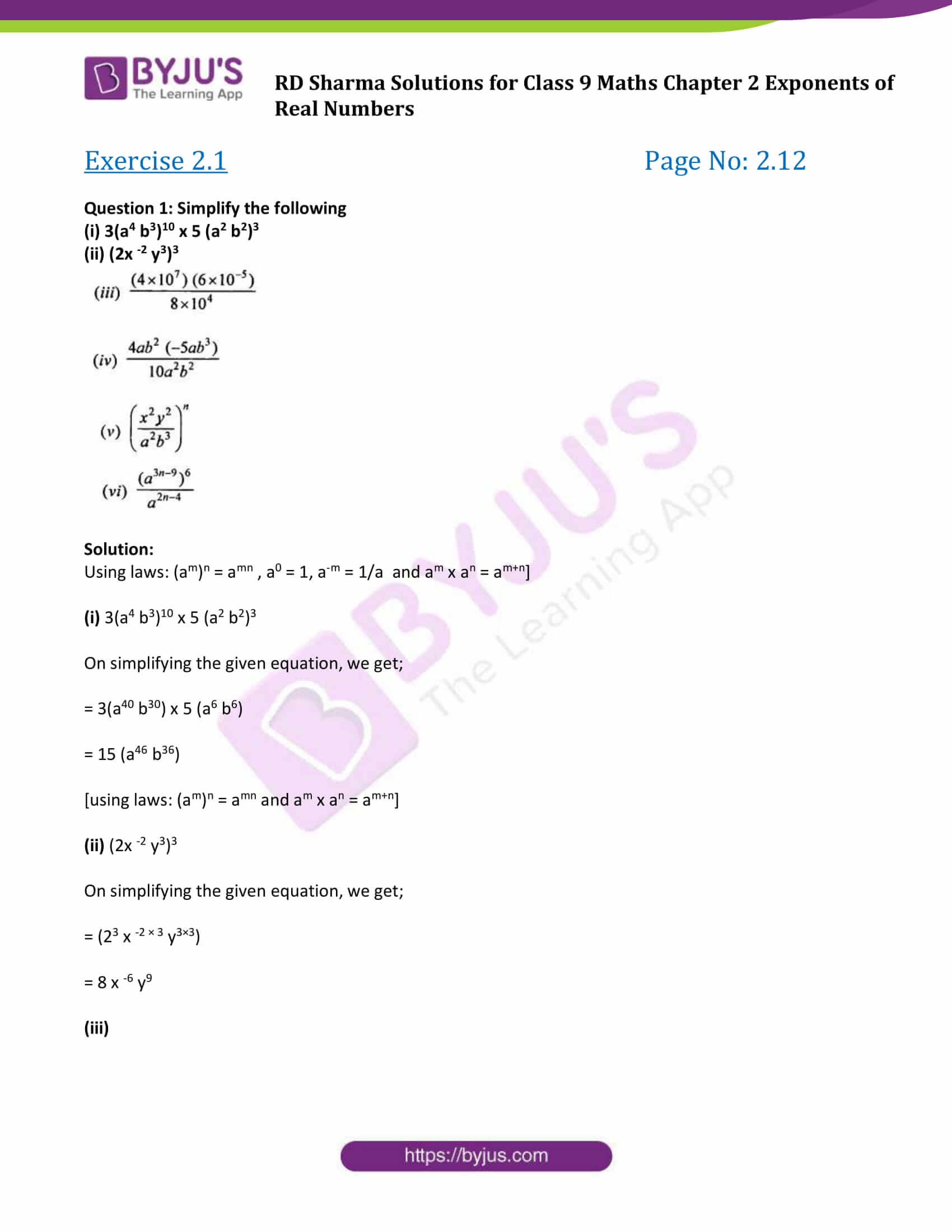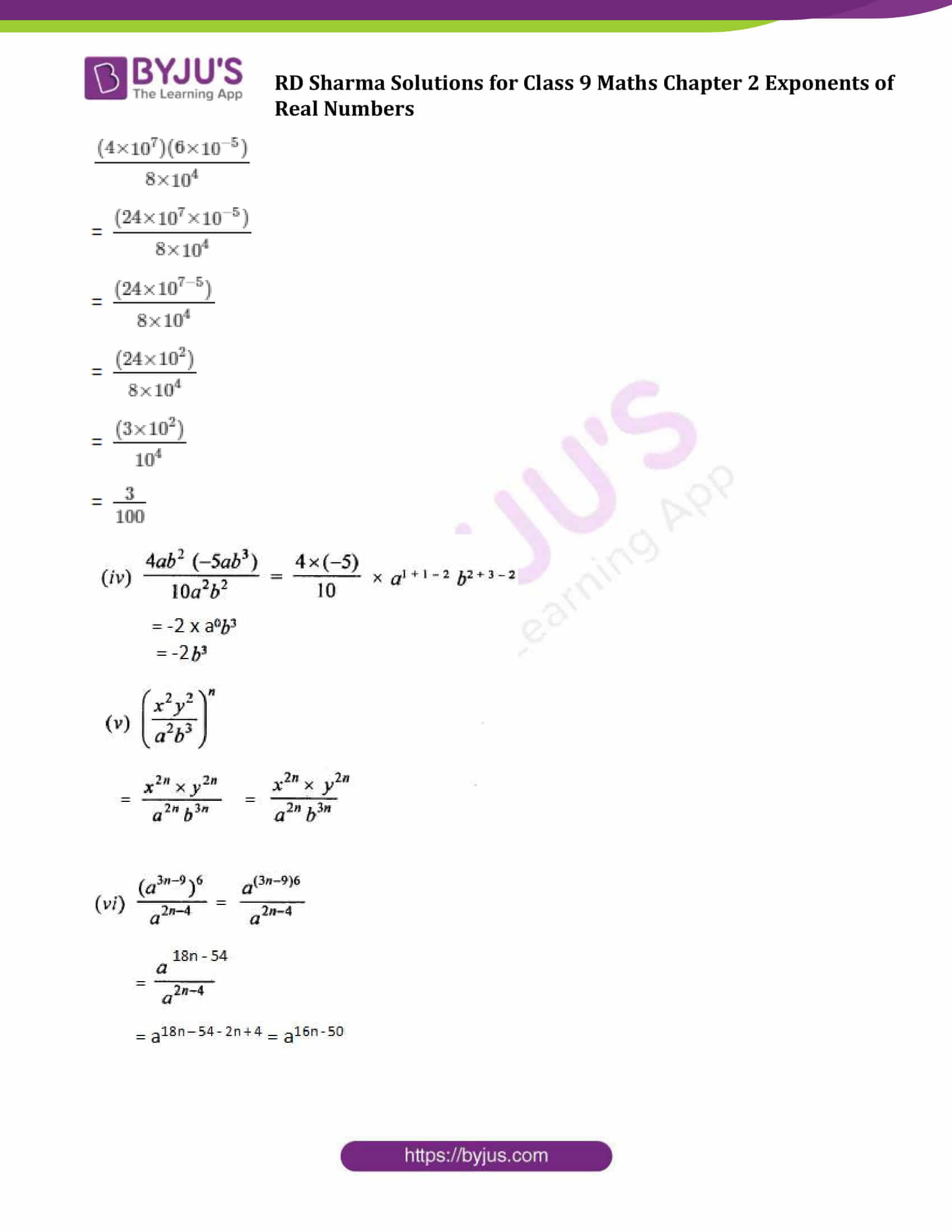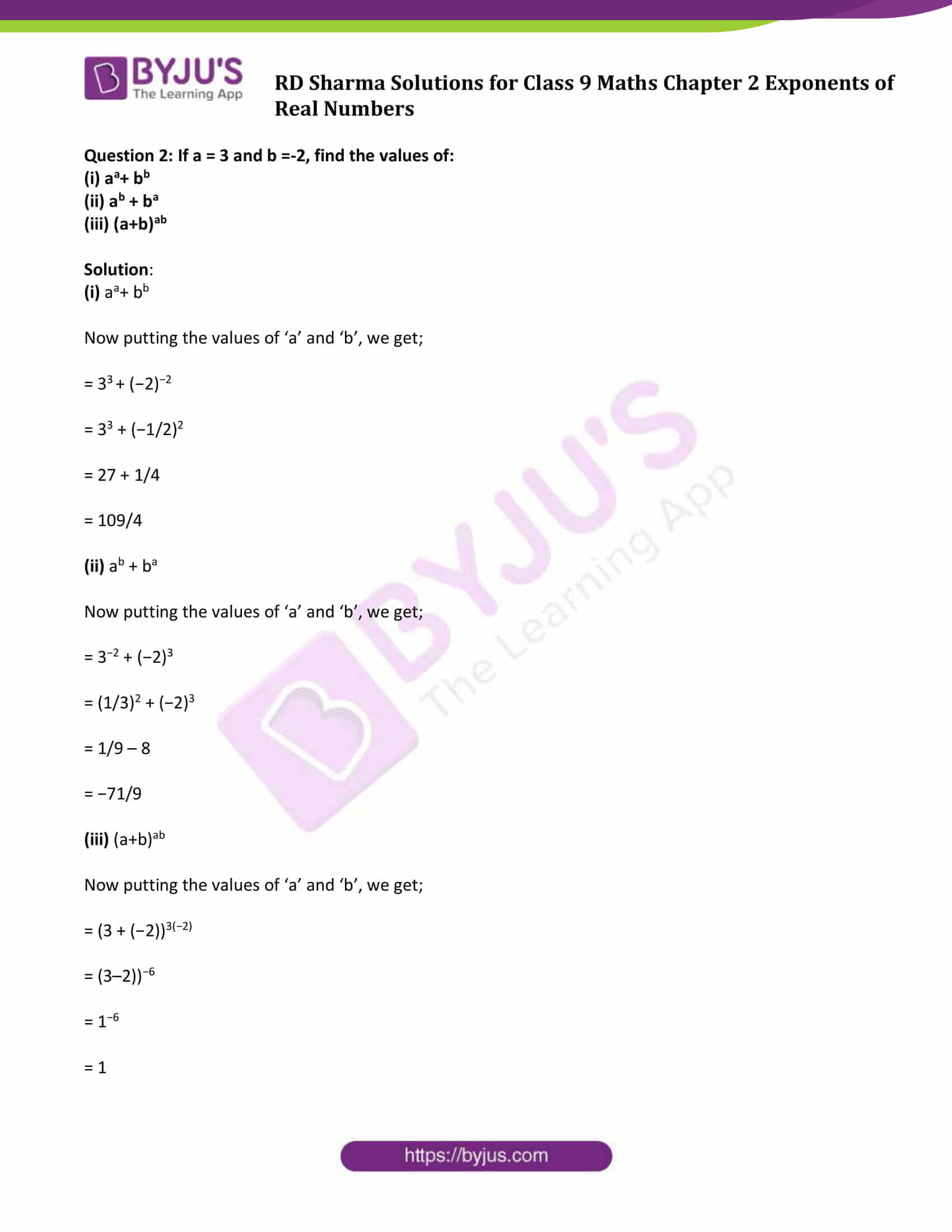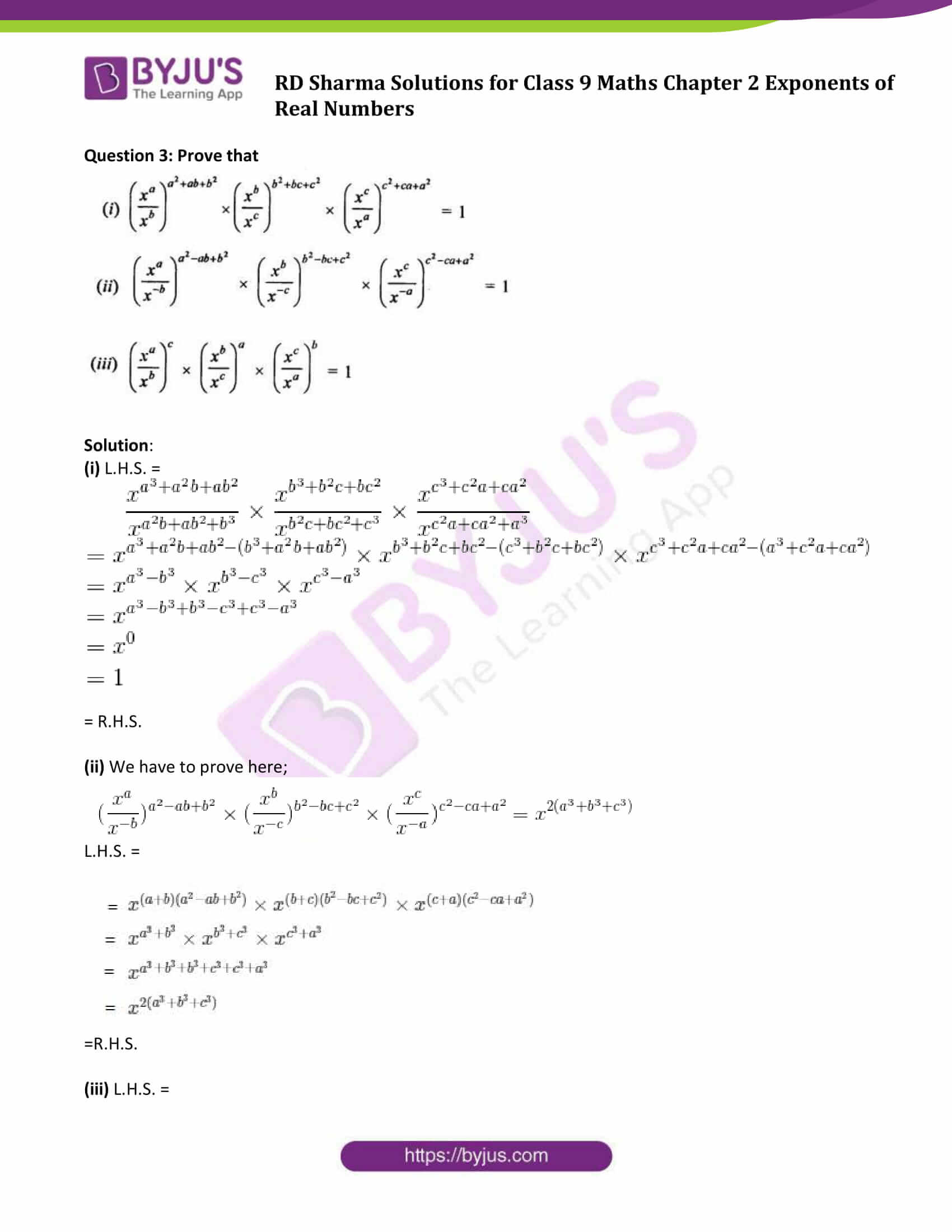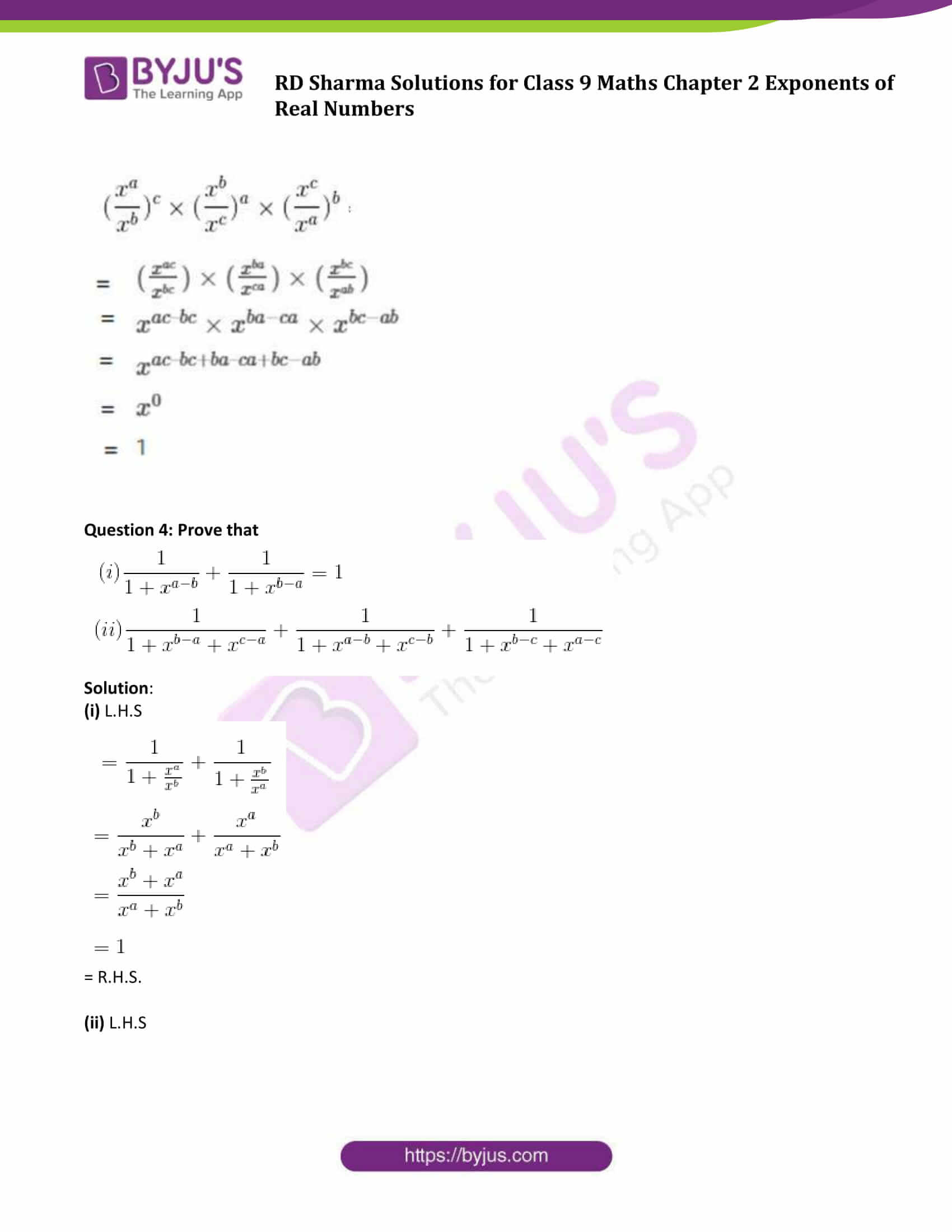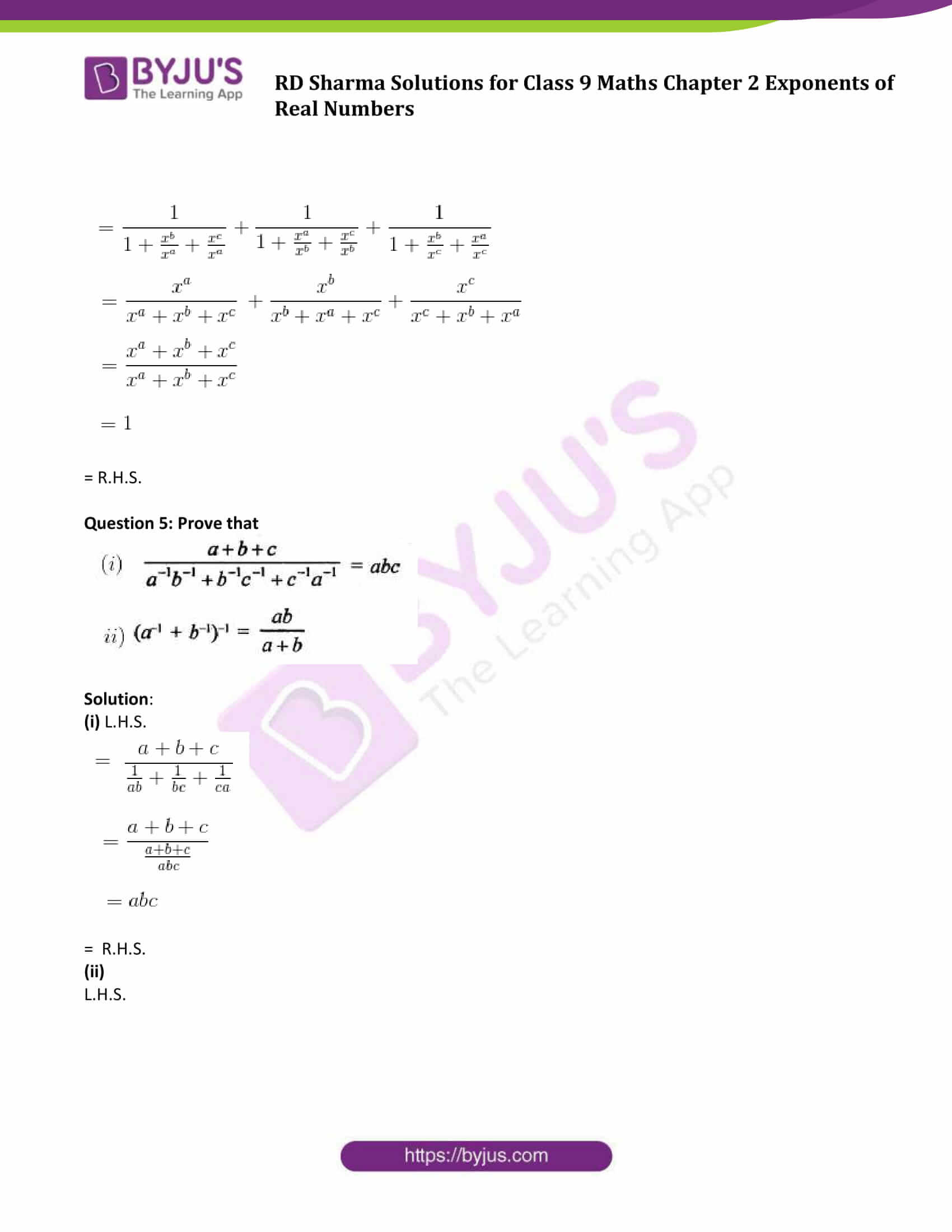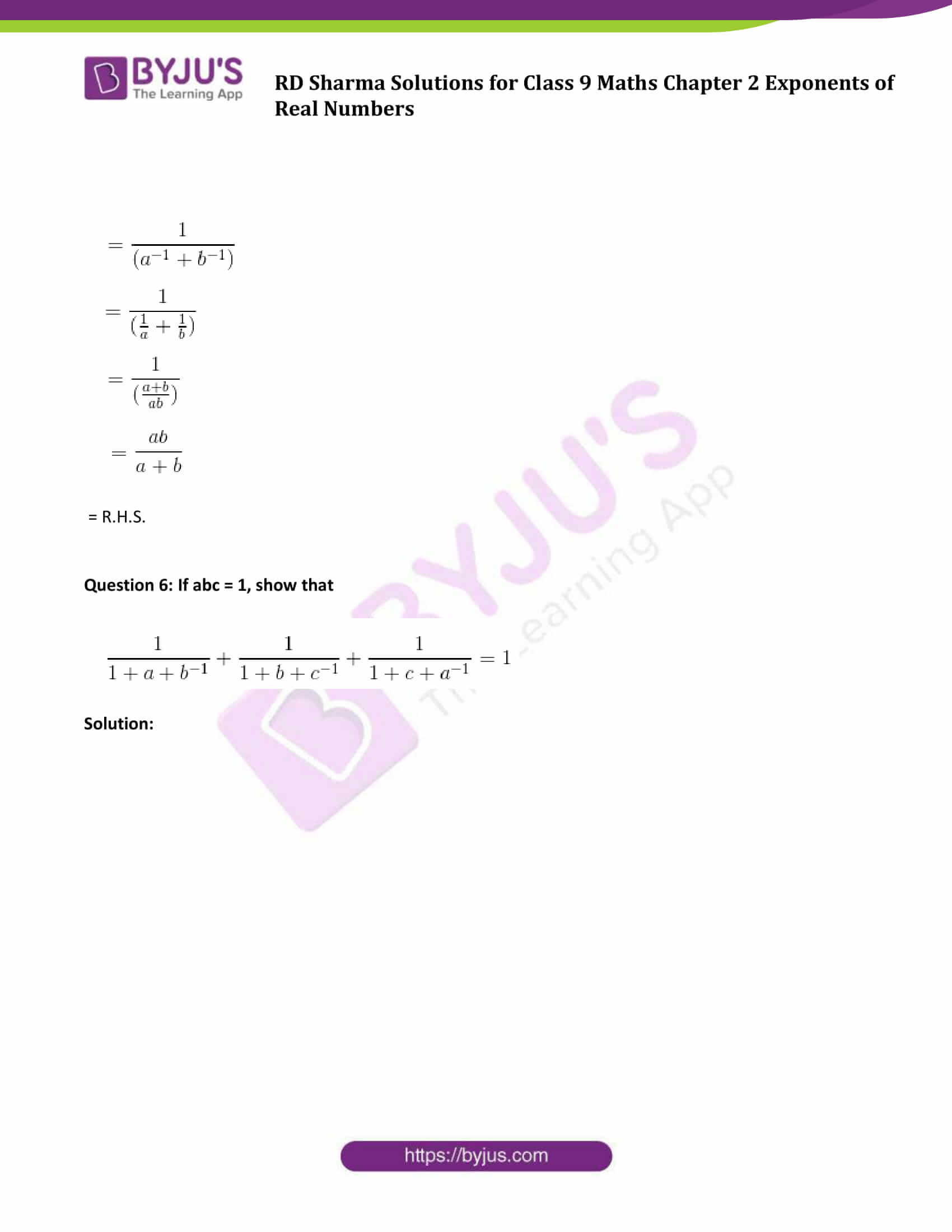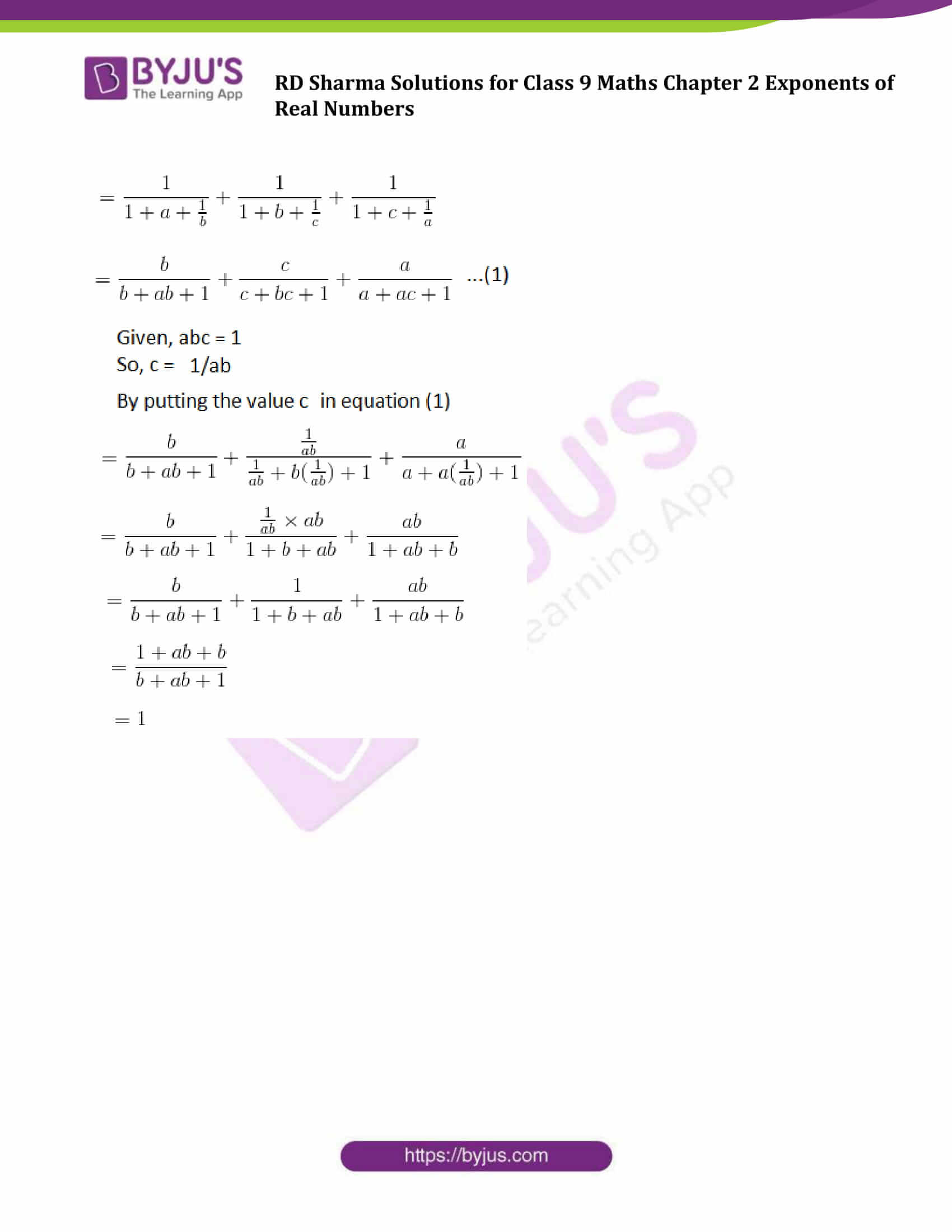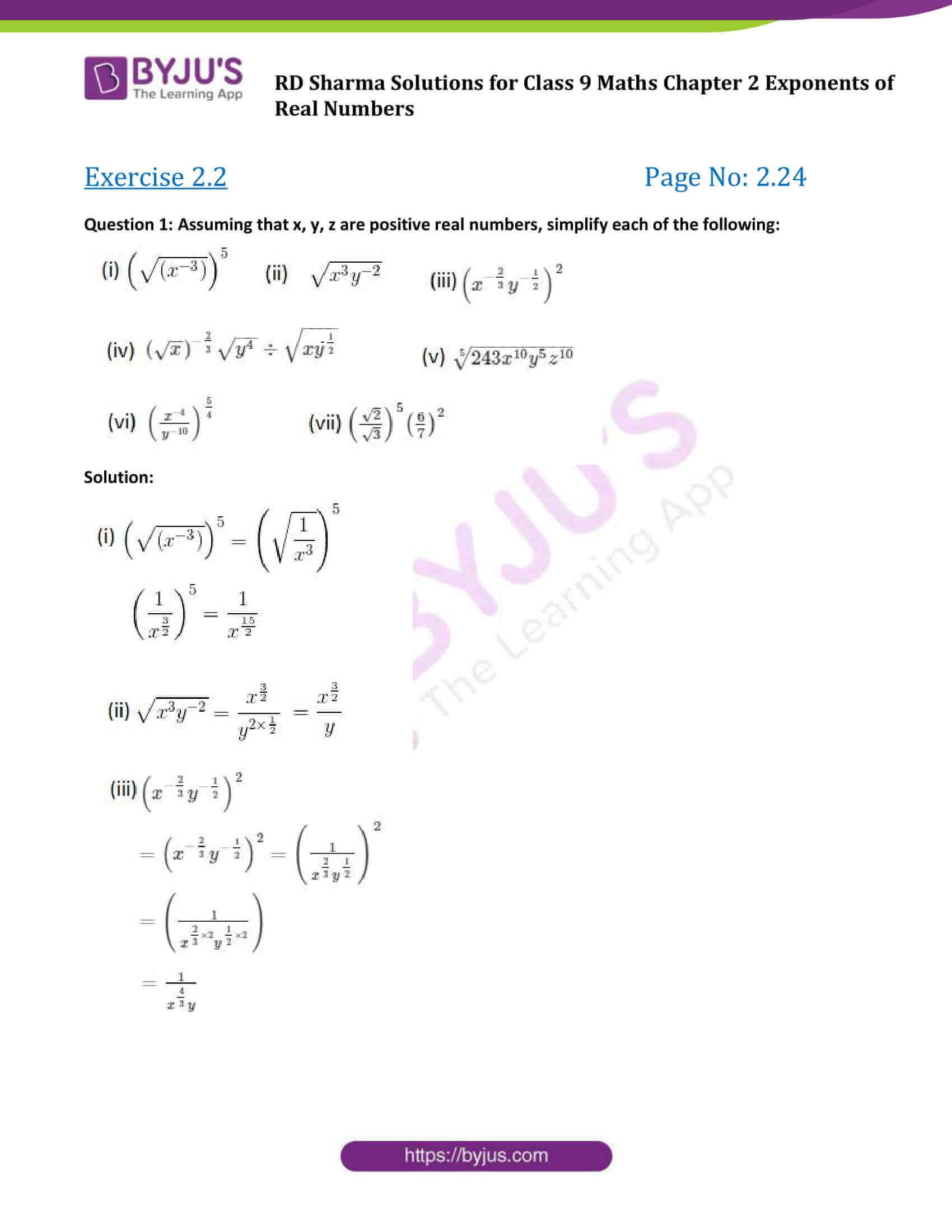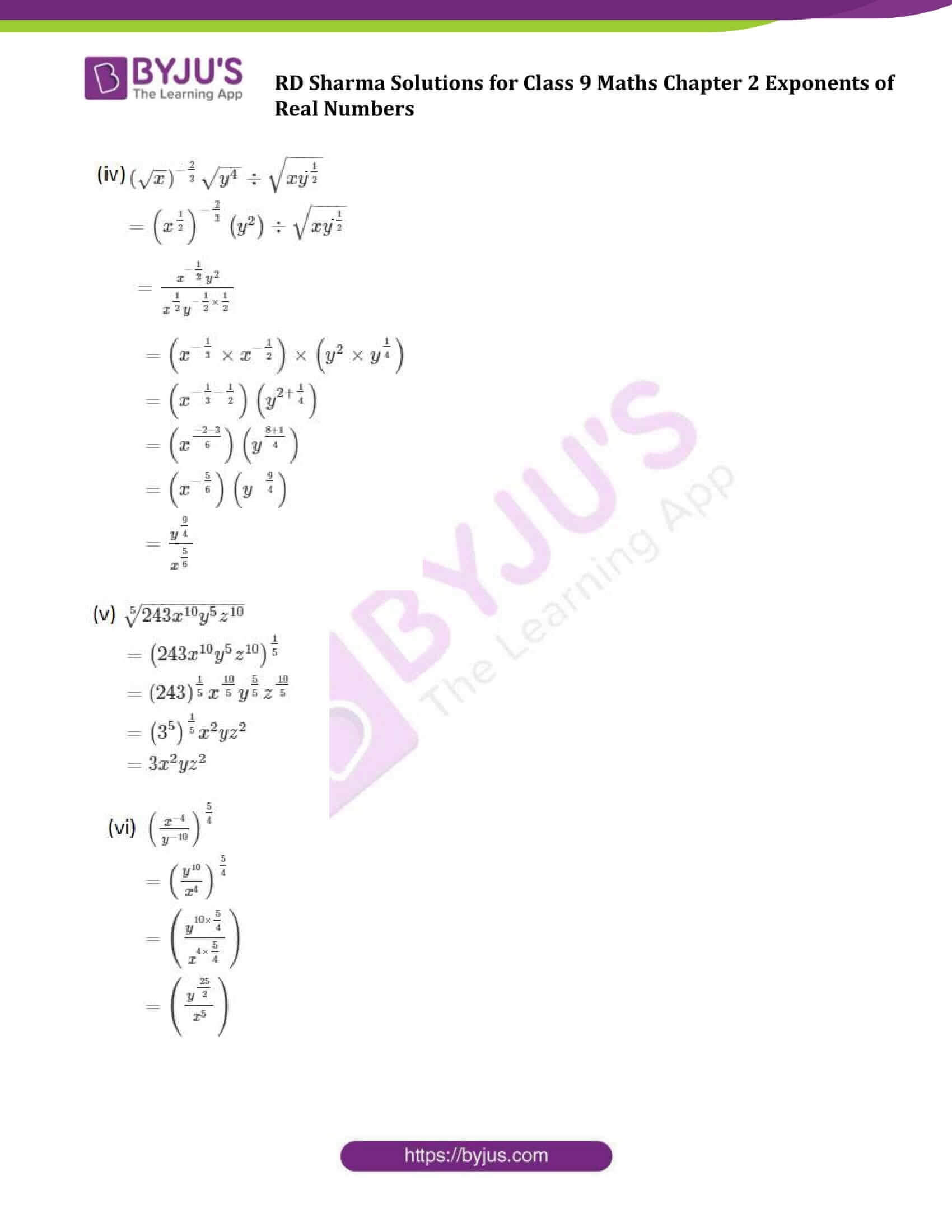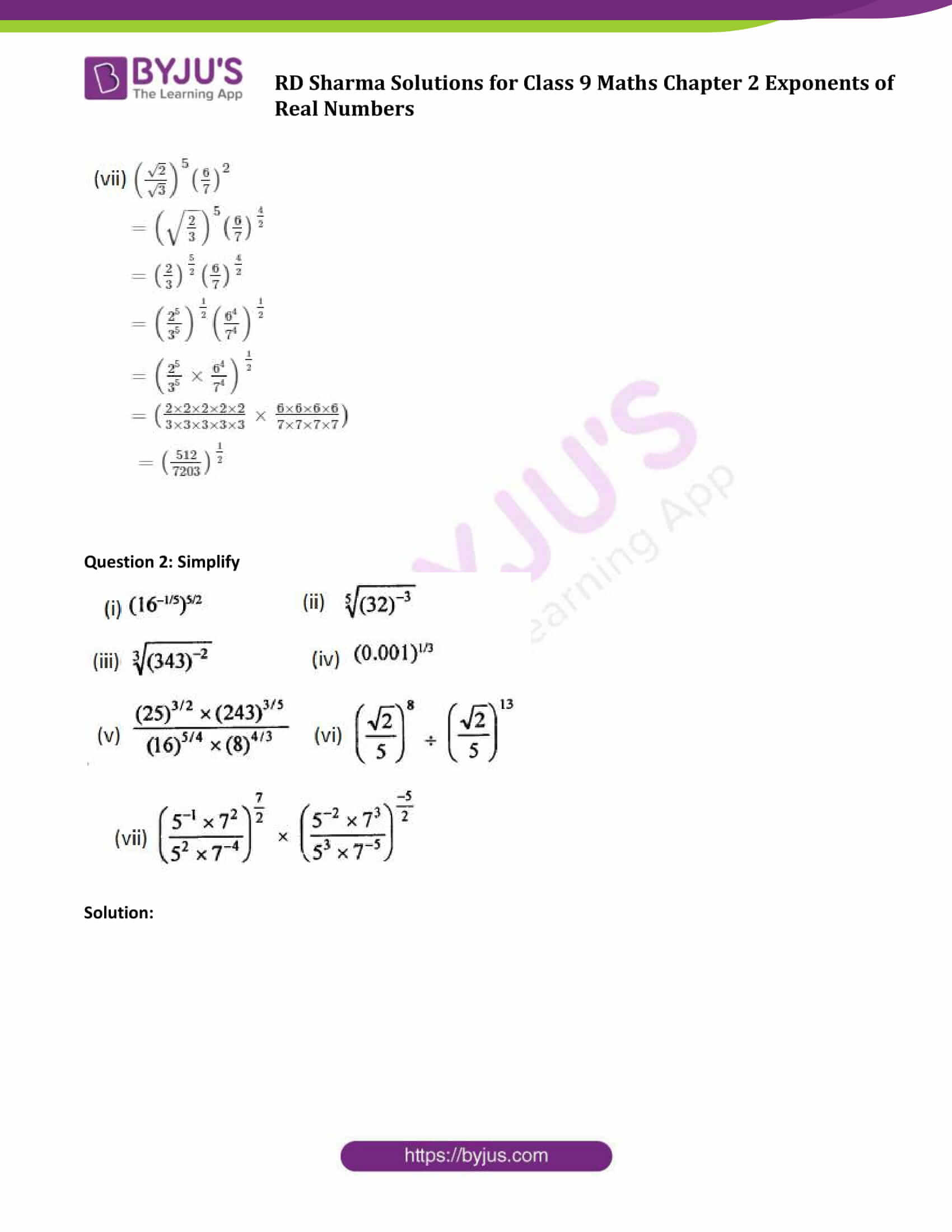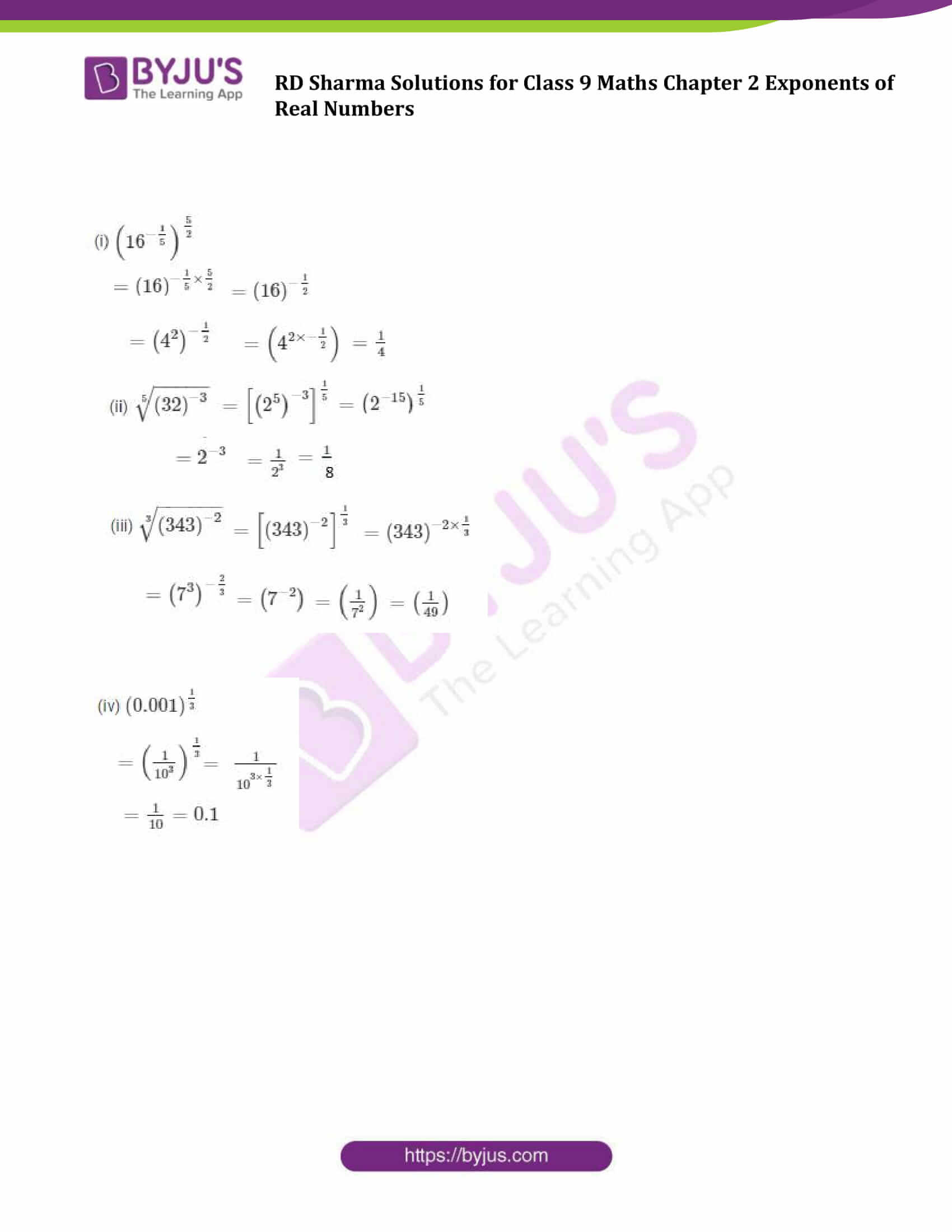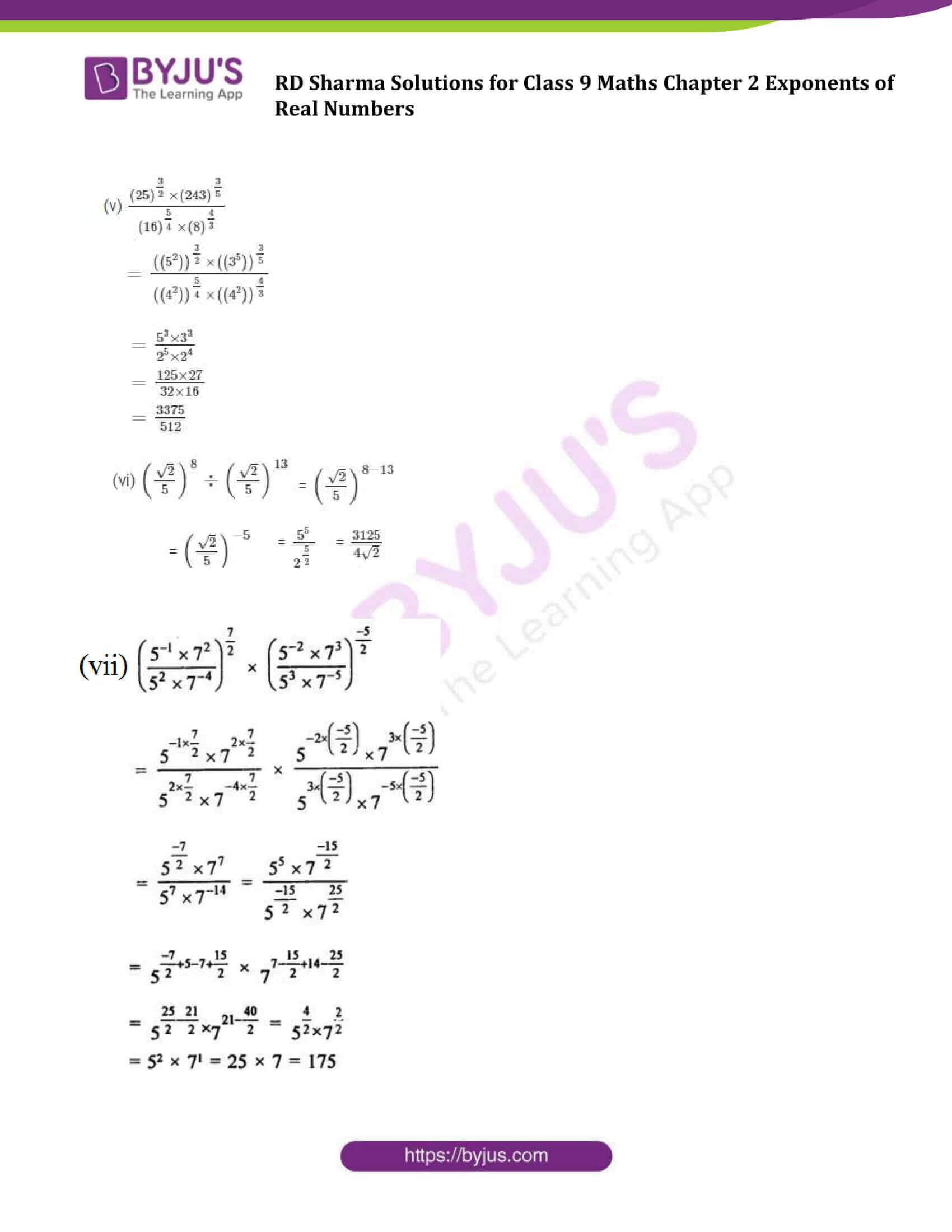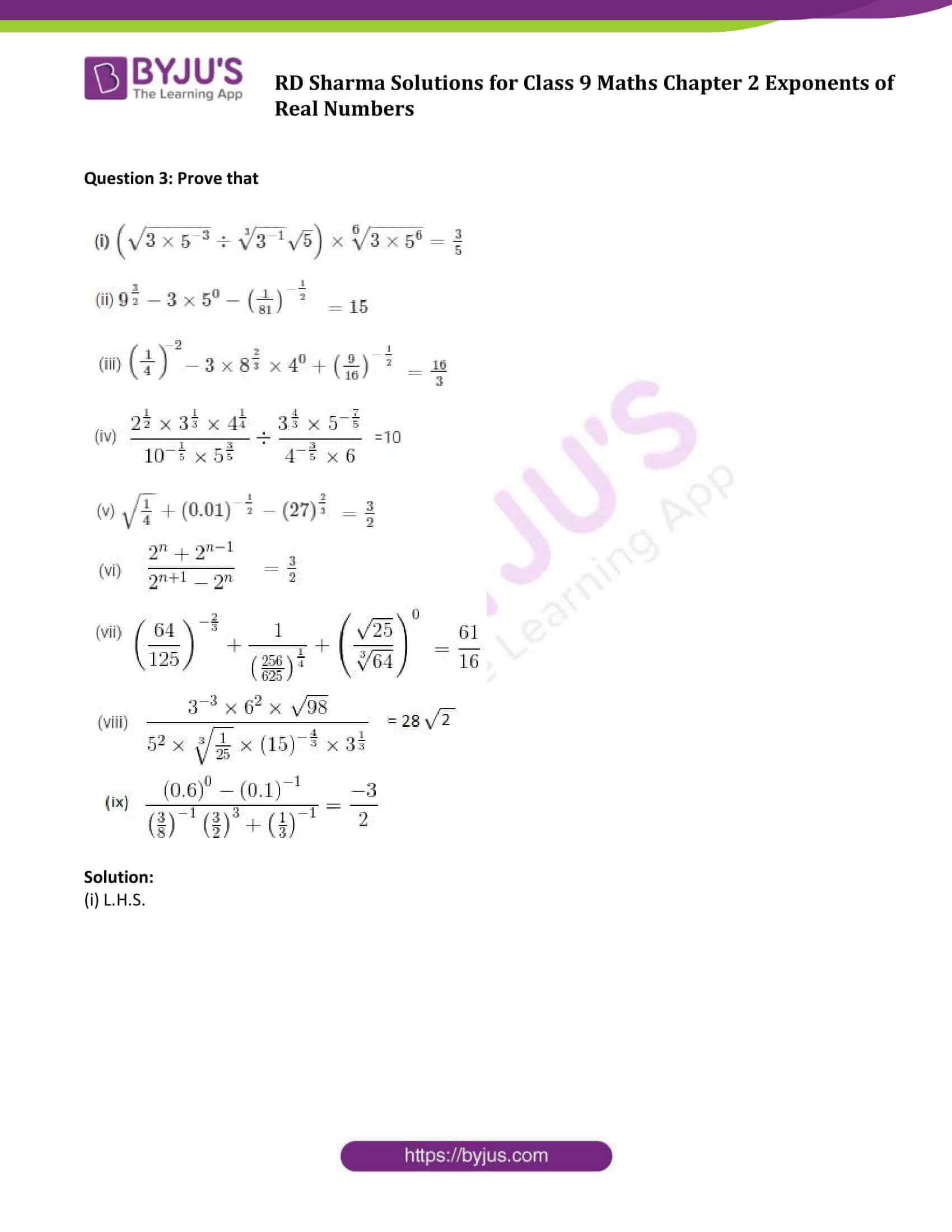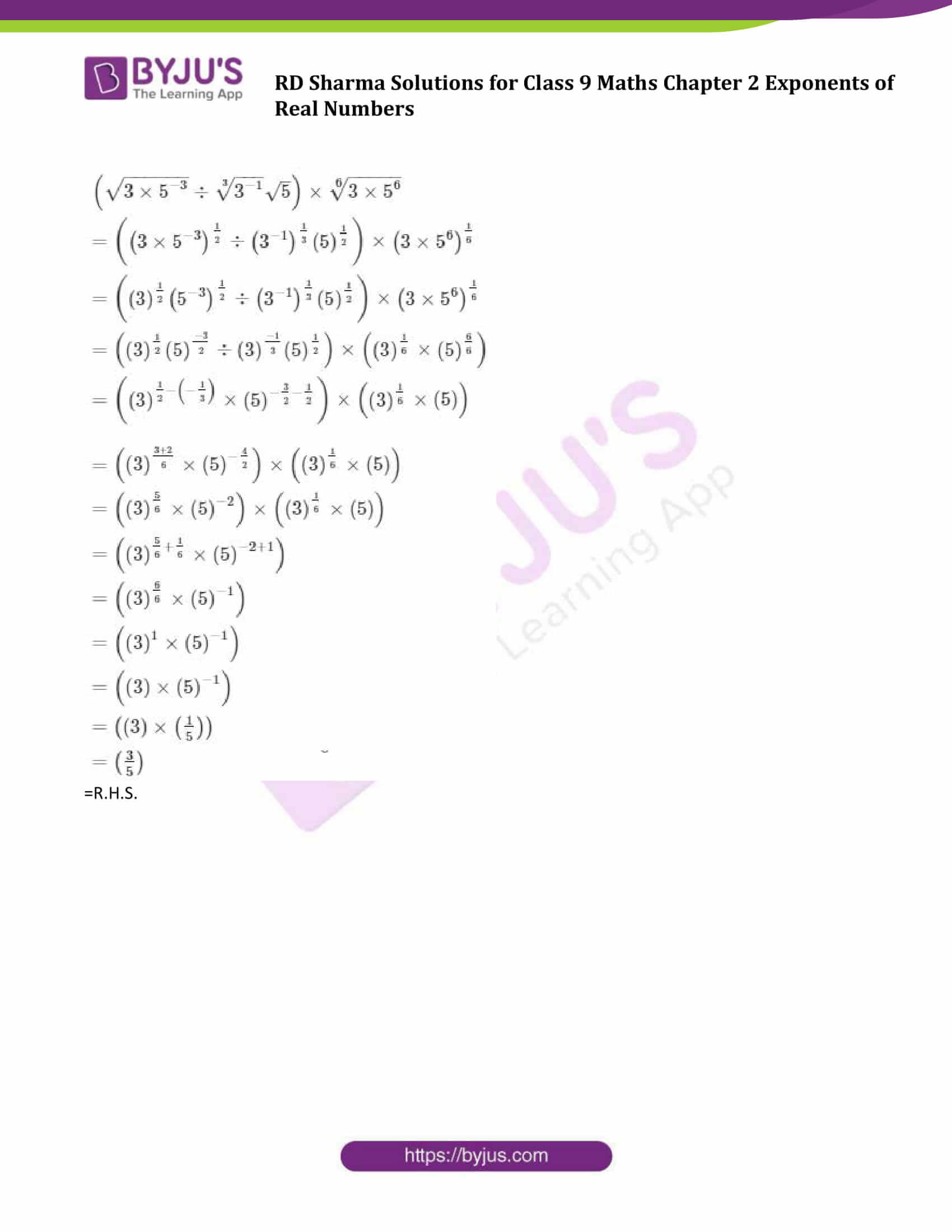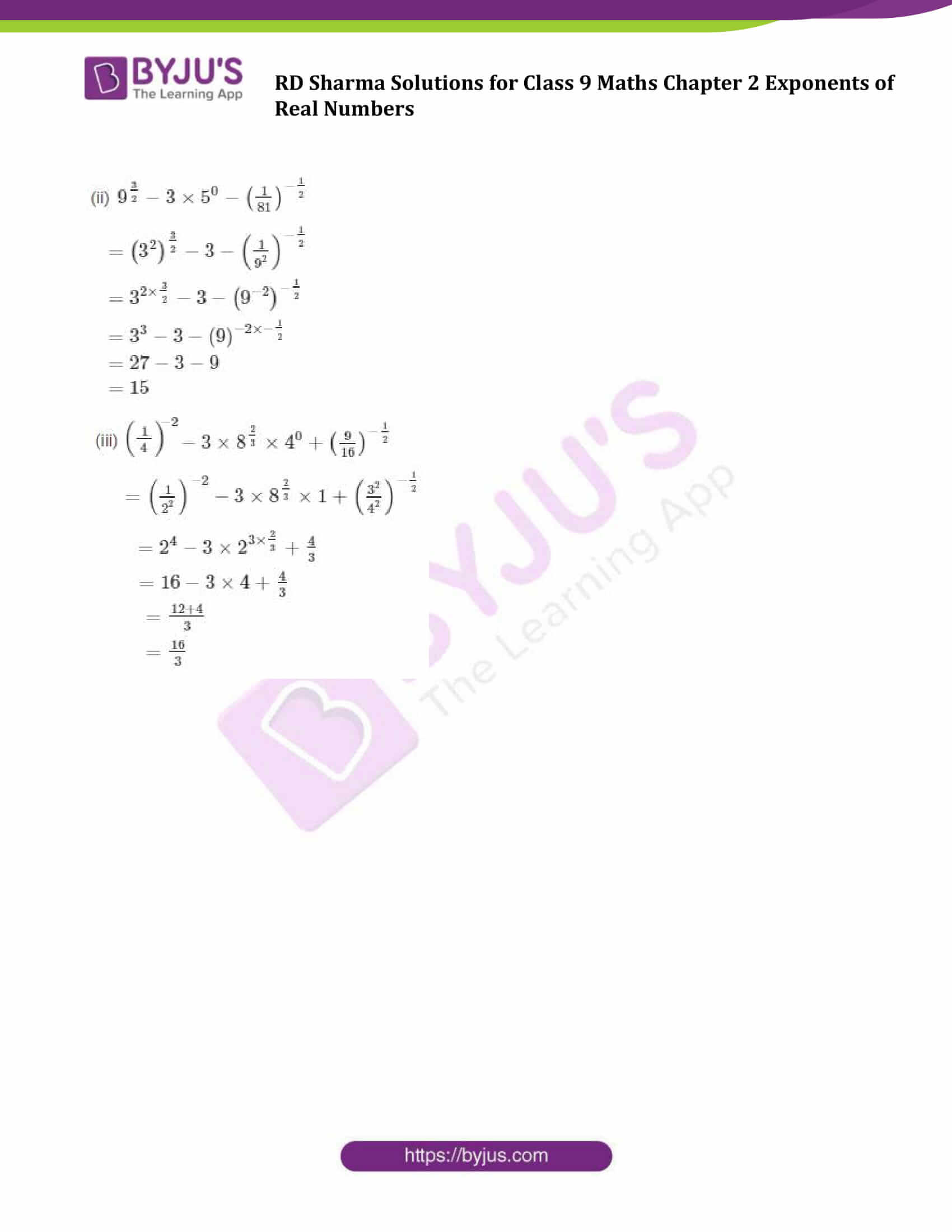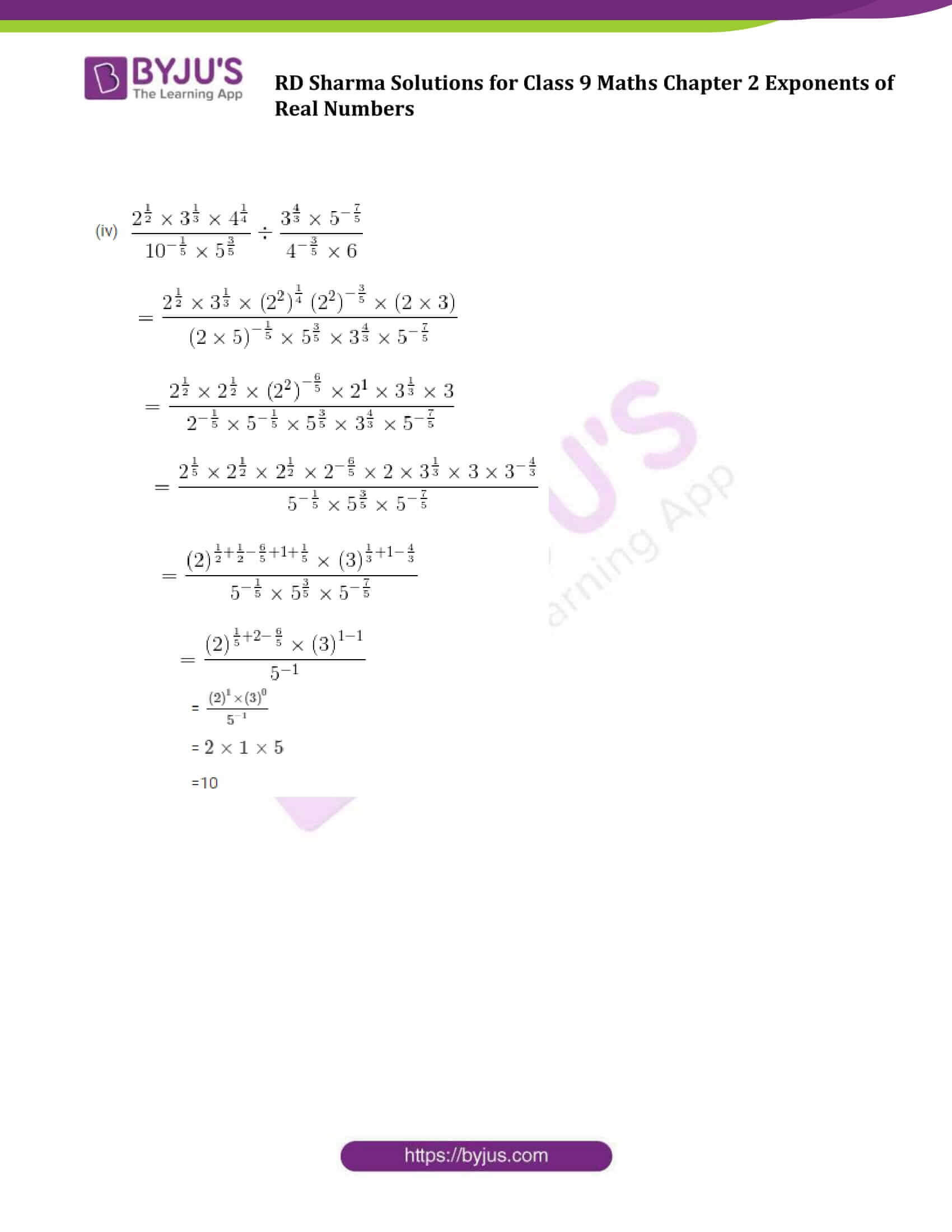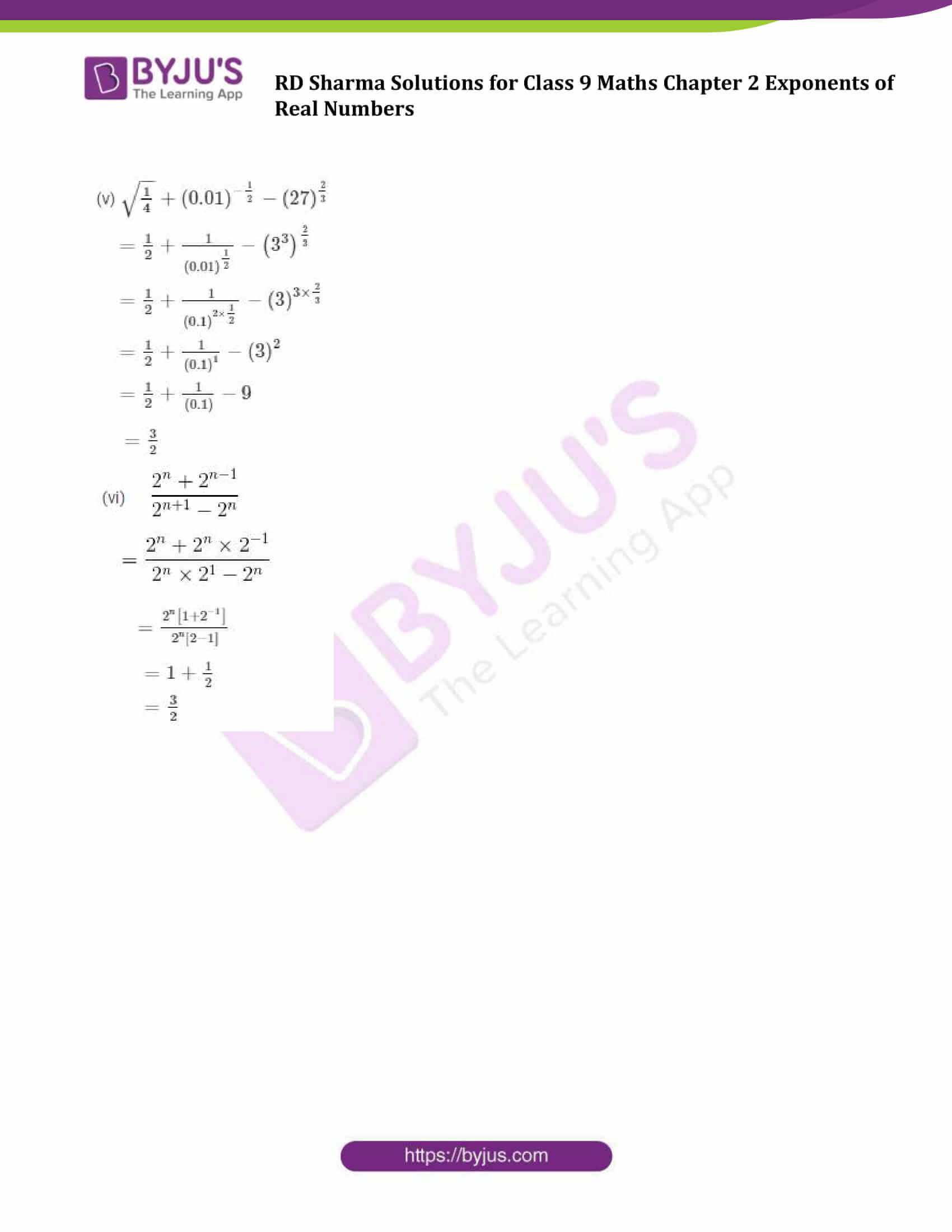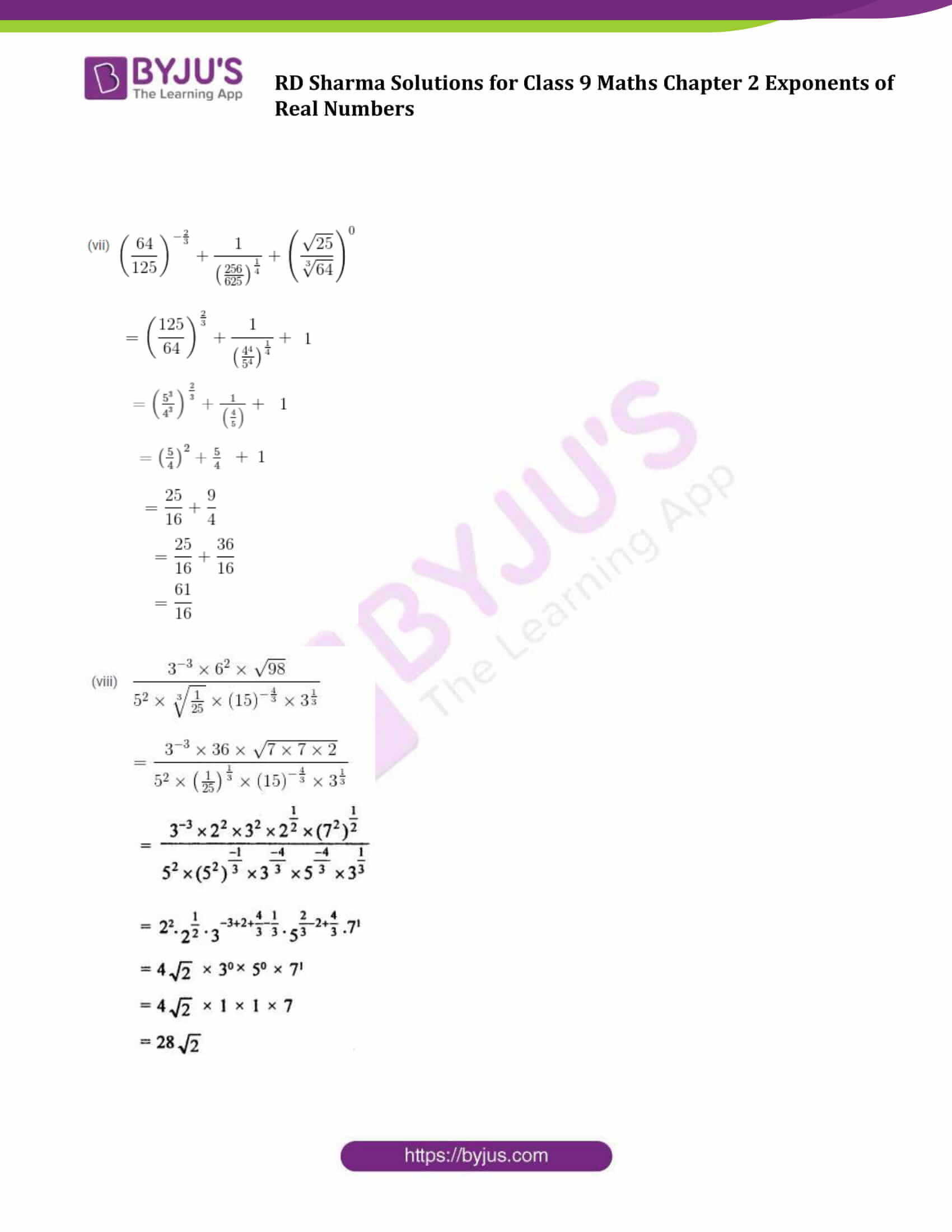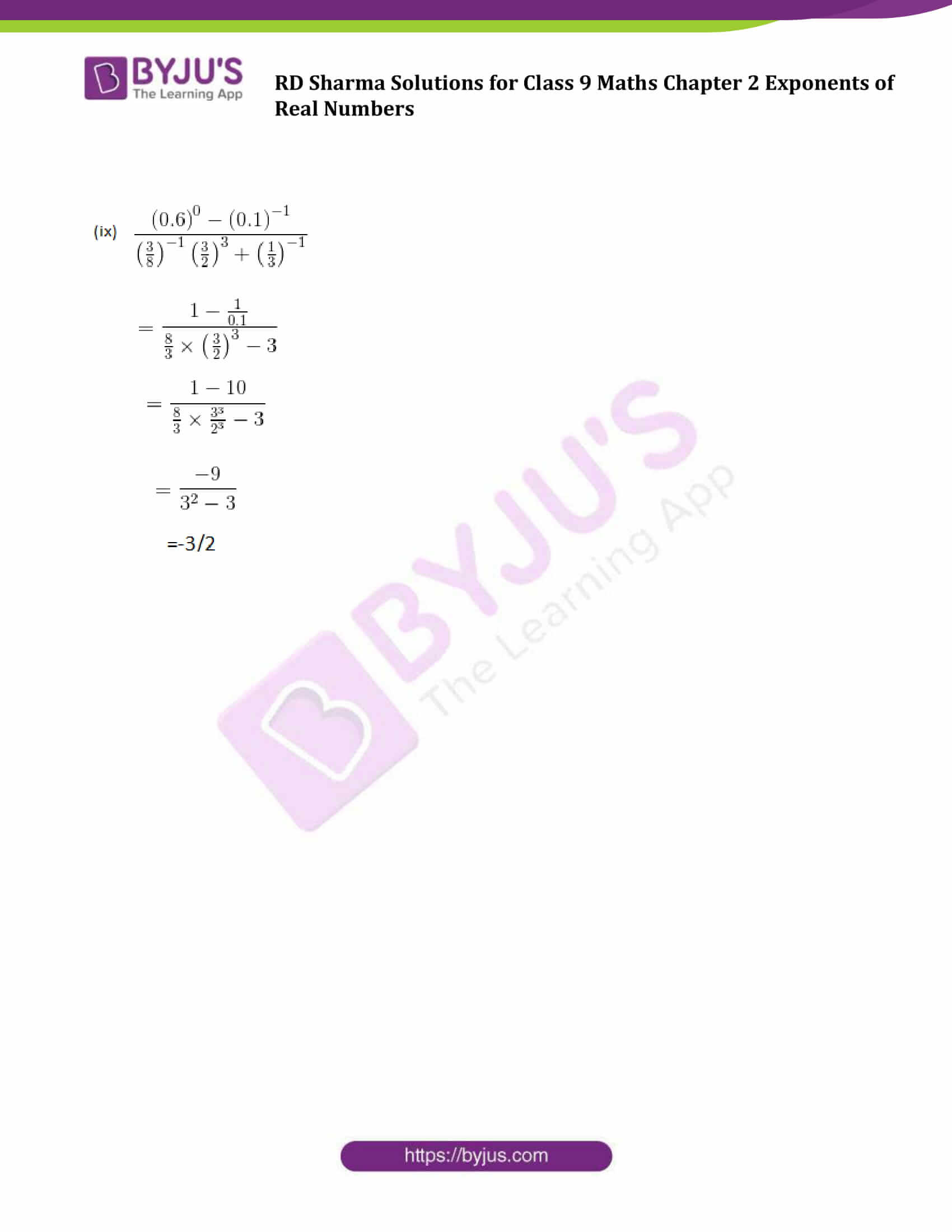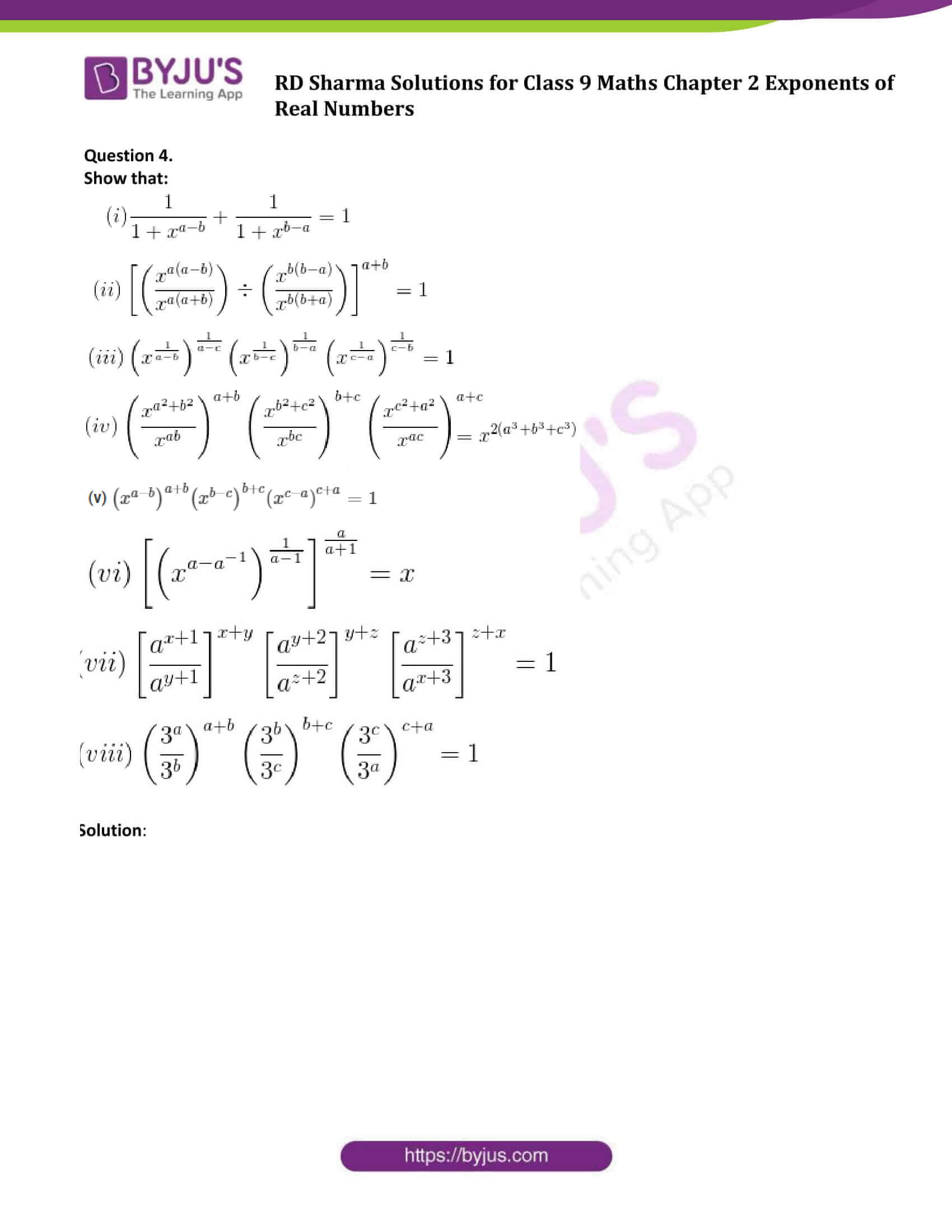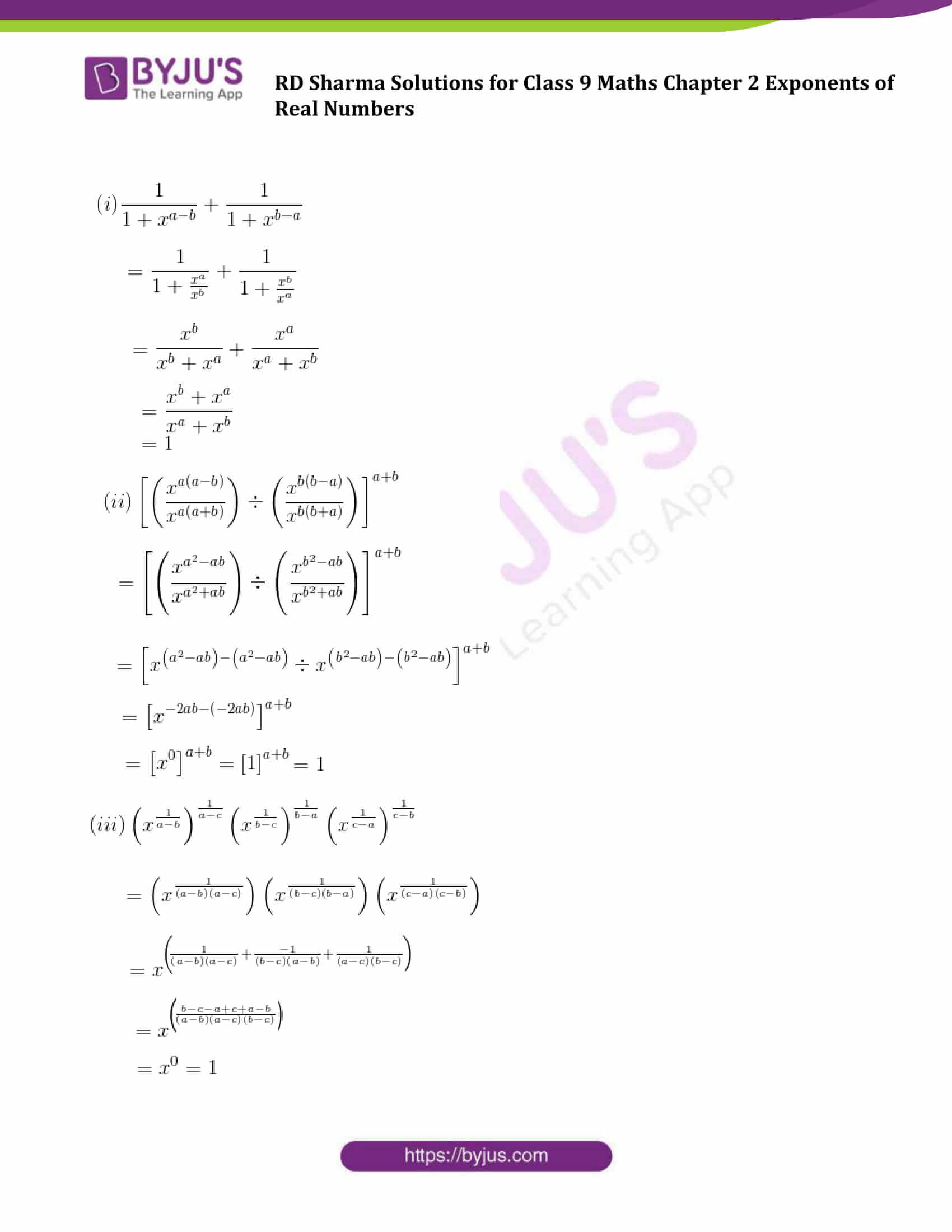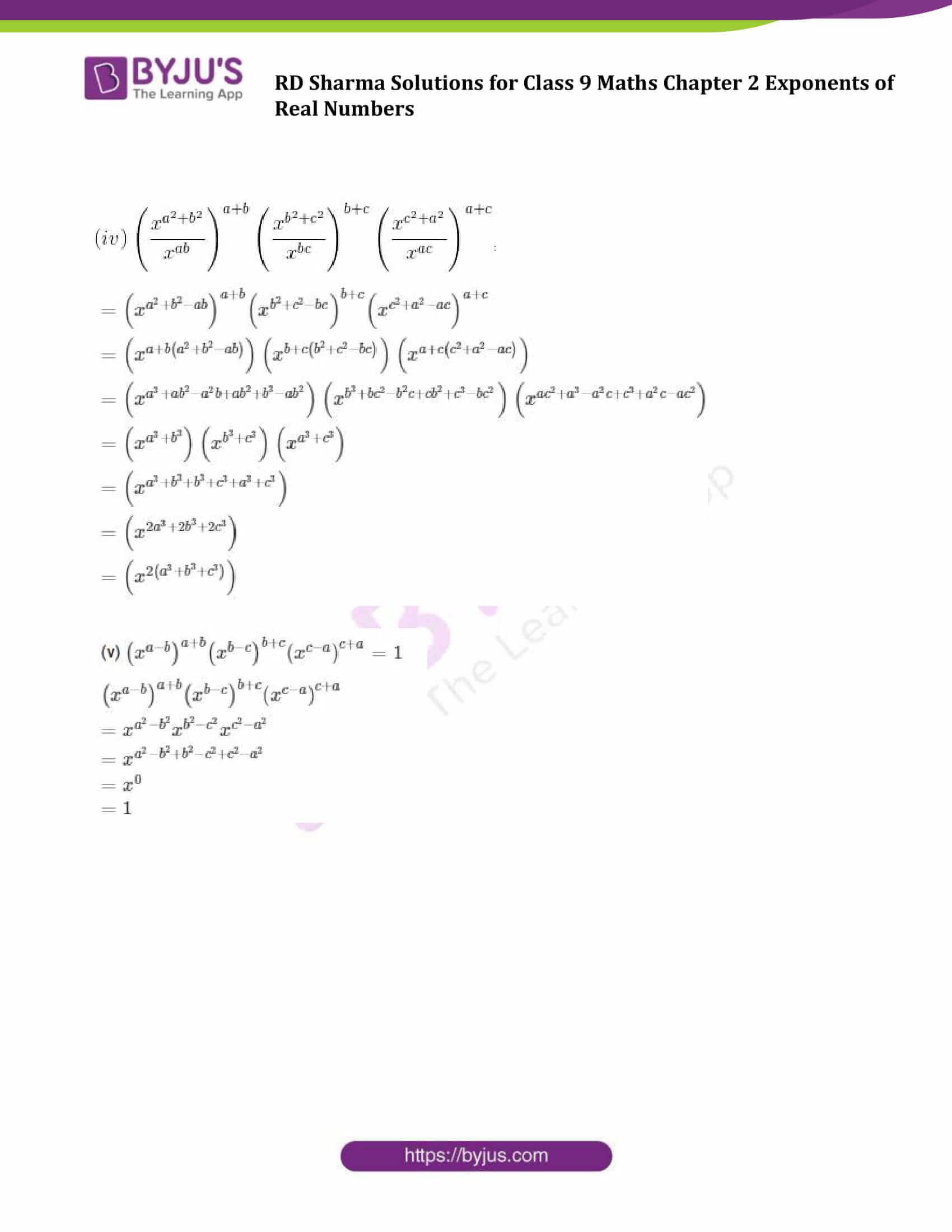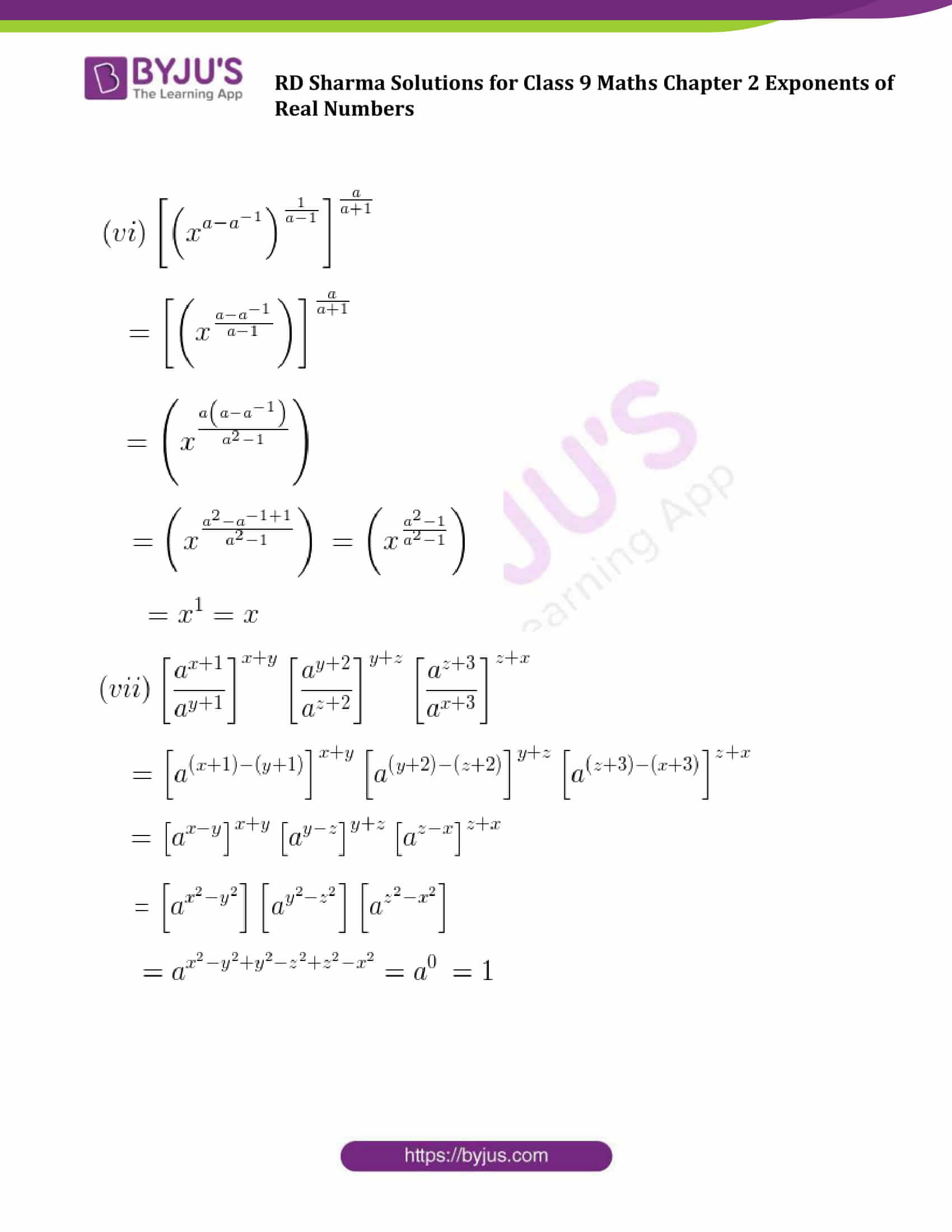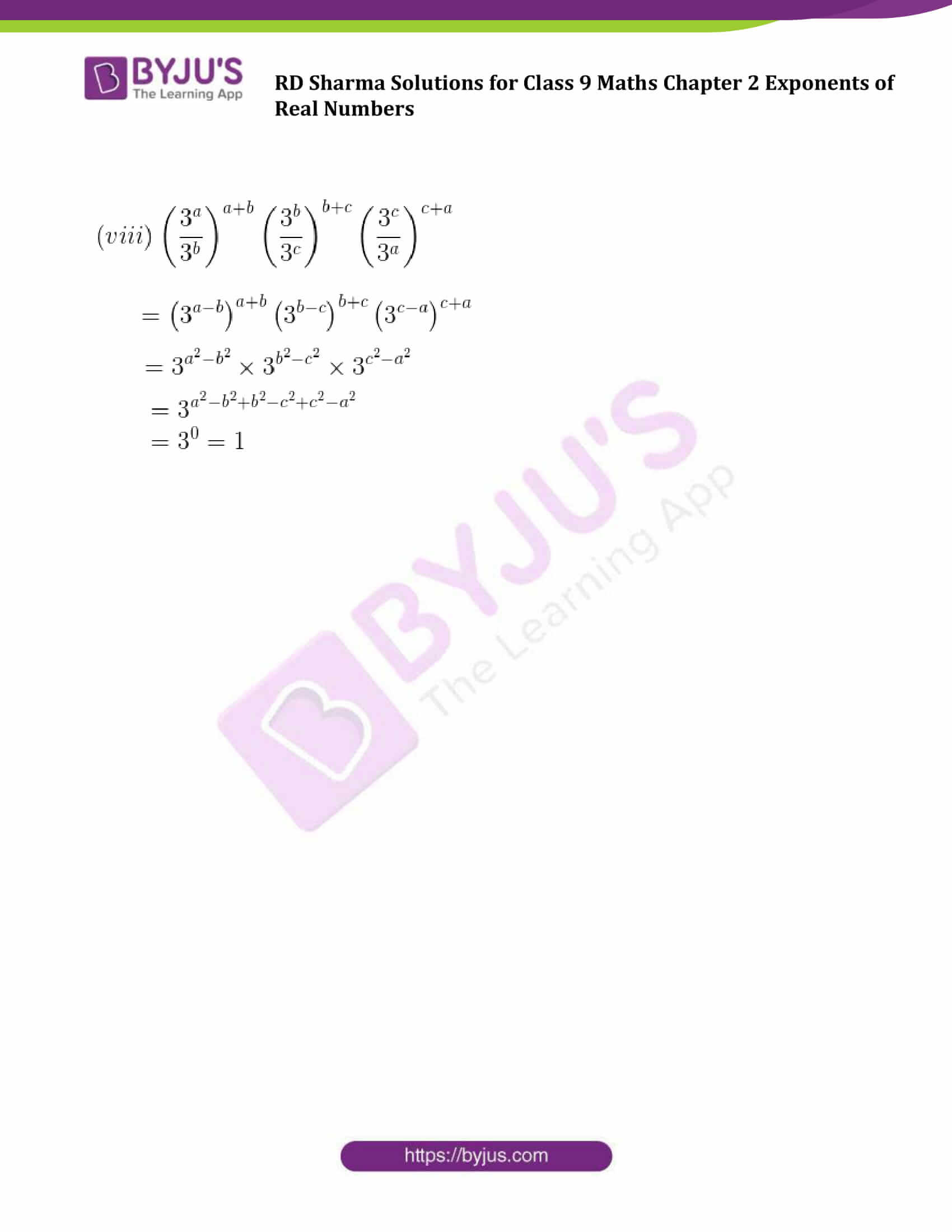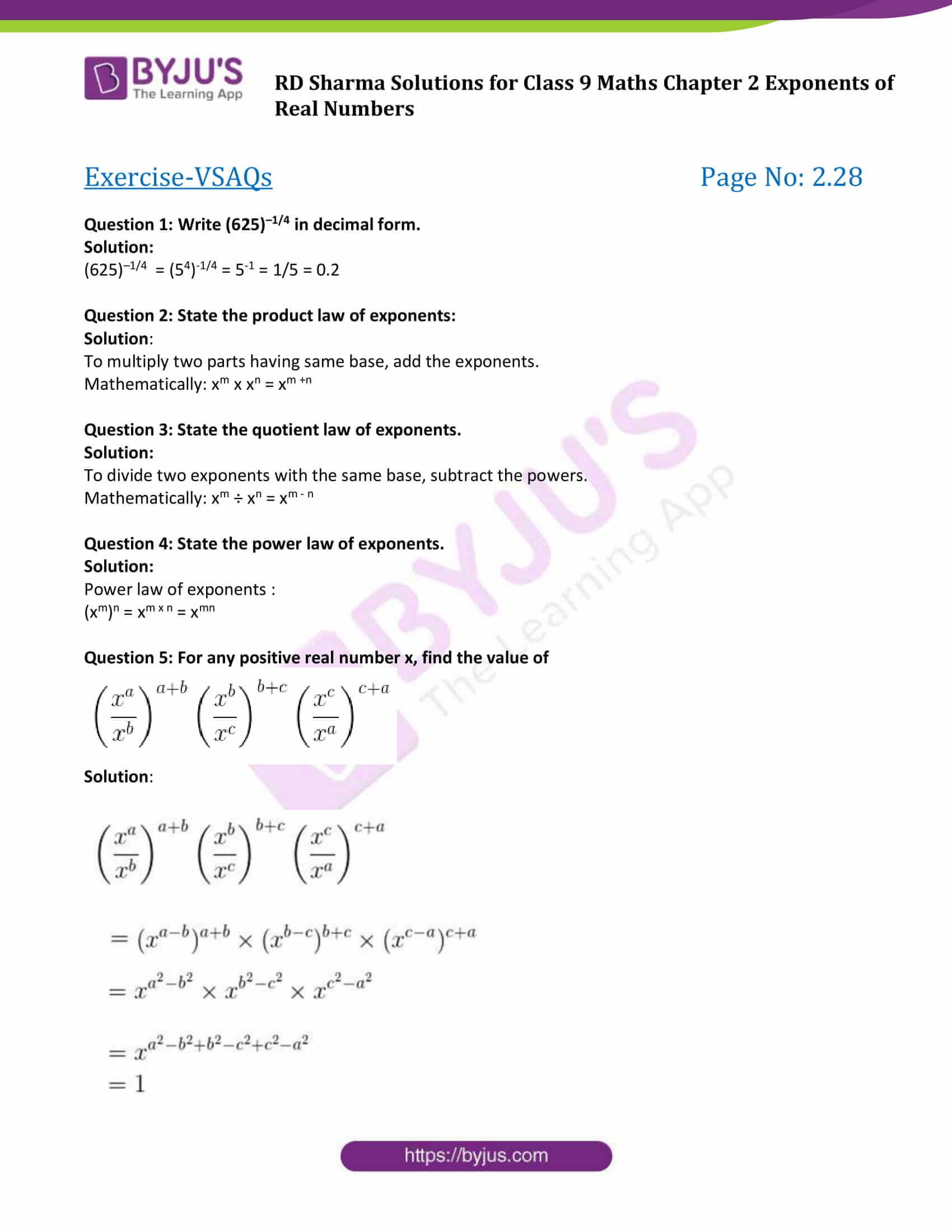### Exercise 2.1

Question 1: Simplify the following

(i) 3(a4 b3)10 x 5 (a2 b2)3

(ii) (2x -2 y3)3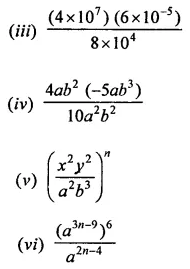Solution:

Using laws: (am)n = amn , a0 = 1, a-m = 1/a and am x an = am+n]

(i) 3(a4 b3)10 x 5 (a2 b2)3

On simplifying the given equation, we get;

= 3(a40 b30) x 5 (a6 b6)

= 15 (a46 b36)

[using laws: (am)n = amn and am x an = am+n]

(ii) (2x -2 y3)3

On simplifying the given equation, we get;

= (23 x -2 × 3 y3×3)

= 8 x -6 y9

(iii)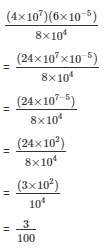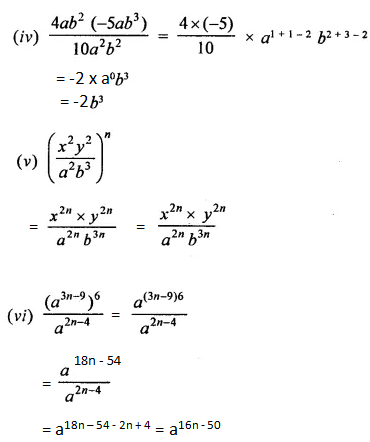Question 2: If a = 3 and b =-2, find the values of:

(i) aa+ bb

(ii) ab + ba

(iii) (a+b)ab

Solution:

(i) aa+ bb

Now putting the values of ‘a’ and ‘b’, we get;

= 33 + (−2)−2

= 33 + (−1/2)2

= 27 + 1/4

= 109/4

(ii) ab + ba

Now putting the values of ‘a’ and ‘b’, we get;

= 3−2 + (−2)3

= (1/3)2 + (−2)3

= 1/9 – 8

= −71/9

(iii) (a+b)ab

Now putting the values of ‘a’ and ‘b’, we get;

= (3 + (−2))3(−2)

= (3–2))−6

= 1−6

= 1

Question 3: Prove that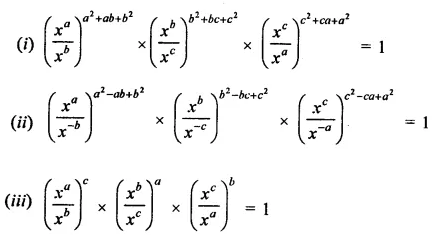Solution:

(i) L.H.S. =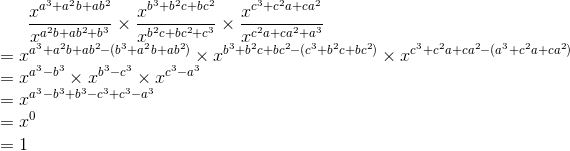= R.H.S.

(ii) We have to prove here;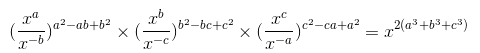L.H.S. =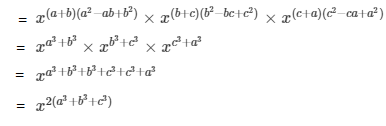=R.H.S.

(iii) L.H.S. =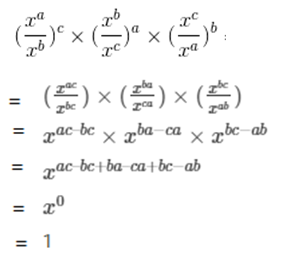= R.H.S.

Question 4: Prove that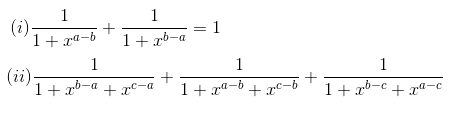Solution:

(i) L.H.S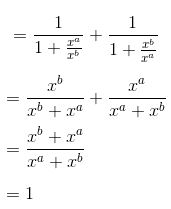= R.H.S.

(ii) L.H.S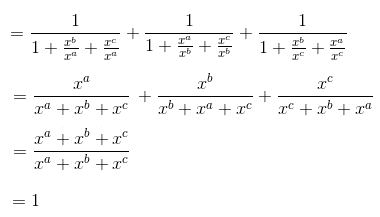= R.H.S.

Question 5: Prove that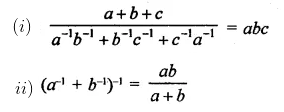Solution:

(i) L.H.S.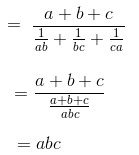= R.H.S.

(ii)

L.H.S.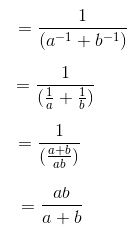= R.H.S.

Question 6: If abc = 1, show that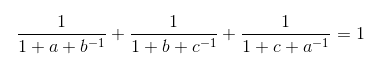Solution: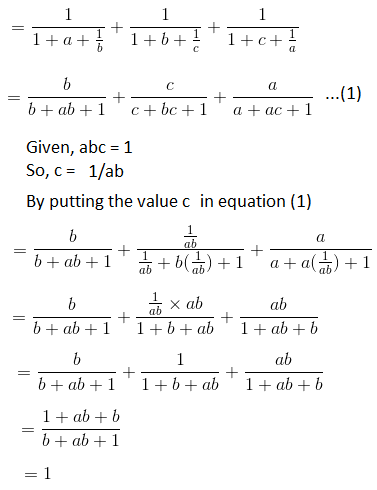### Exercise 2.2

Question 1: Assuming that x, y, z are positive real numbers, simplify each of the following: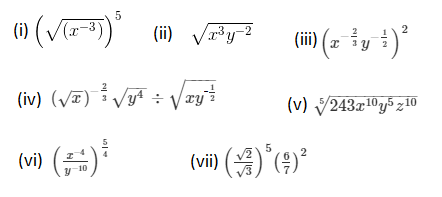Solution: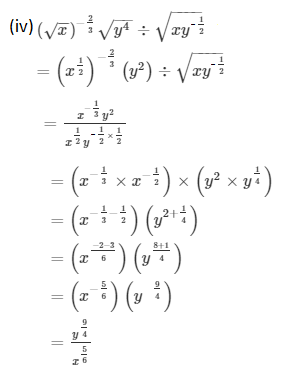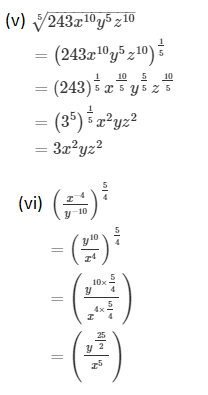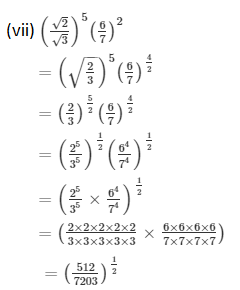Question 2: Simplify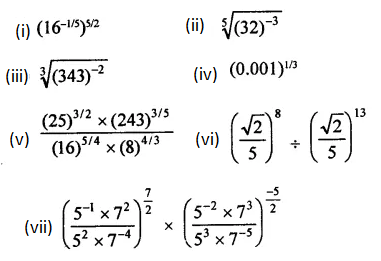Solution: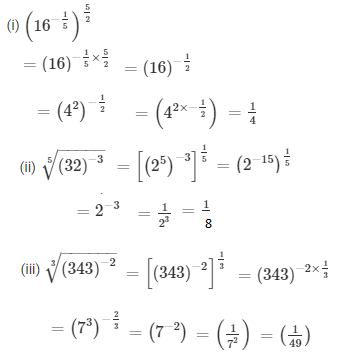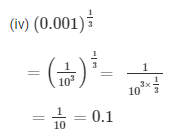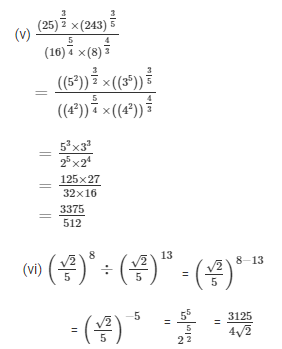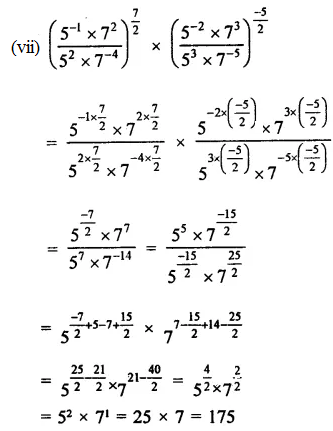Question 3: Prove that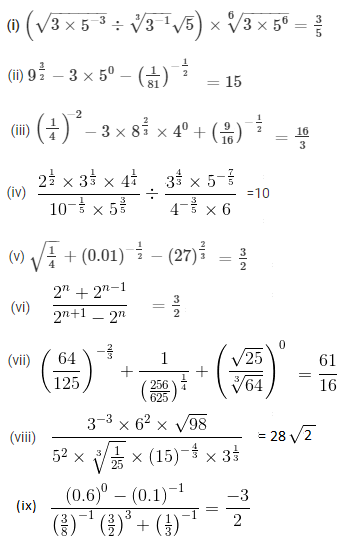Solution:

(i) L.H.S.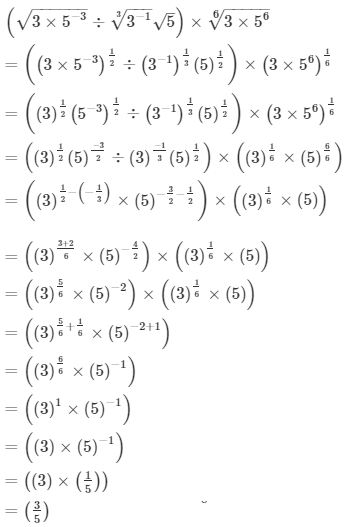=R.H.S.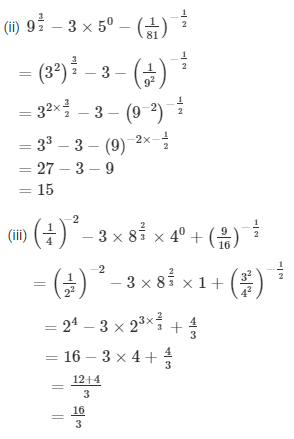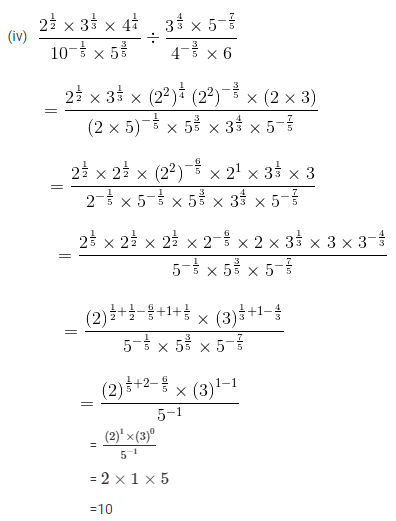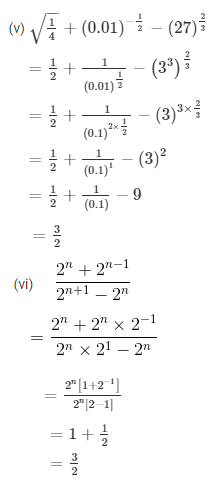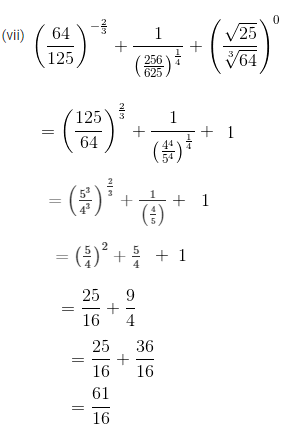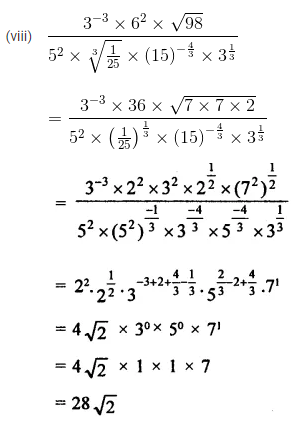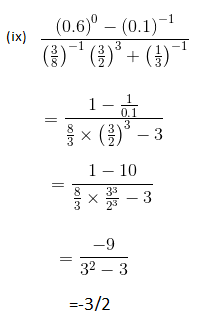Question 4.

Show that: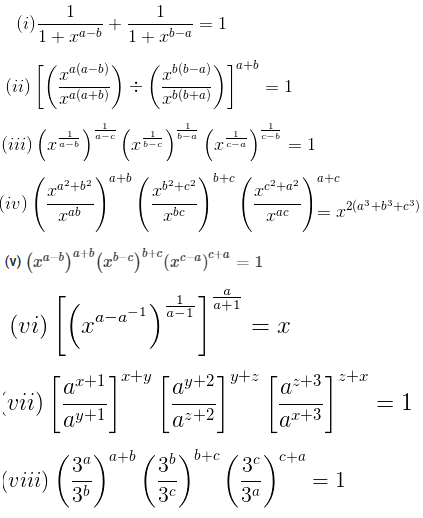Solution: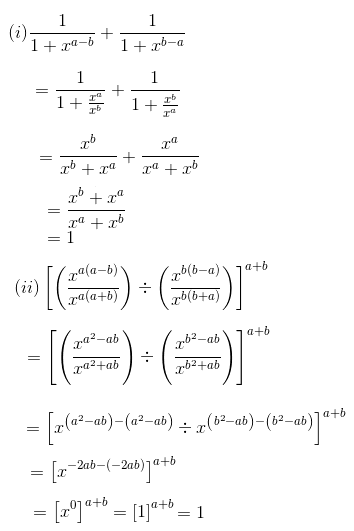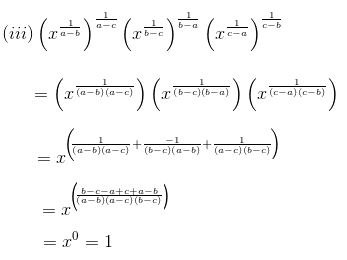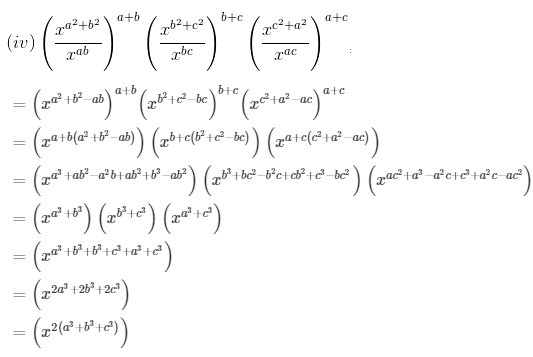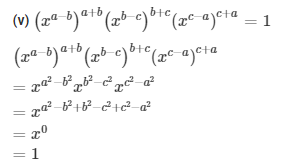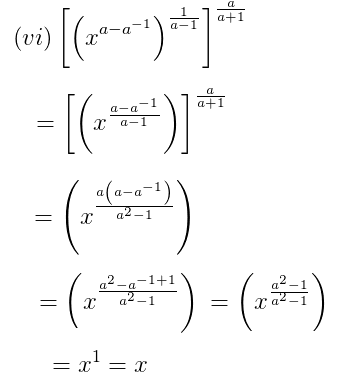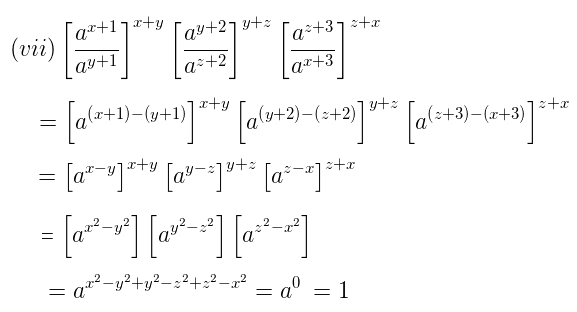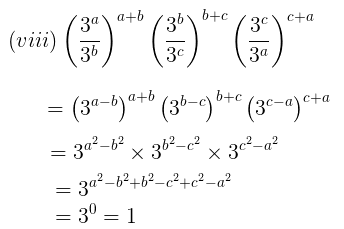### Exercise-VSAQs

Question 1: Write (625)–1/4 in decimal form.

Solution:

(625)–1/4 = (54)-1/4 = 5-1 = 1/5 = 0.2

Question 2: State the product law of exponents:

Solution:

To multiply two parts having same base, add the exponents.

Mathematically: xm x xn = xm +n

Question 3: State the quotient law of exponents.

Solution:

To divide two exponents with the same base, subtract the powers.

Mathematically: xm ÷ xn = xm – n

Question 4: State the power law of exponents.

Solution:

Power law of exponents :

(xm)n = xm x n = xmn

Question 5: For any positive real number x, find the value of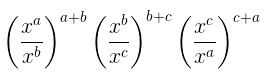Solution: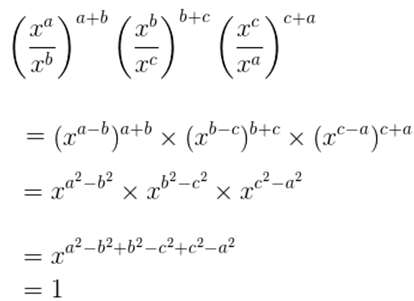Question 6: Write the value of {5(81/3 + 271/3 ) 3}1/4 .

Solution:

{5(81/3 + 271/3 ) 3}1/4

= {5(23×1/3 + 33×1/3 ) 3}1/4

= { 5(2 + 3)^3}1/4

= (54 ) 1/4

= 5

### RD Sharma Solutions for Class 9 Maths Chapter 2 Exponents of Real Numbers

In the 2nd chapter of Class 9 RD Sharma Solutions students will study important concepts on Exponents of Real Numbers as listed below:

• Exponents of Real Numbers Introduction
• Integral exponents of a Real Number
• Laws of Integral Exponents
• Rational Exponents of Real Number
• nth root of a positive Real Number
• Laws of rational exponents

To know these concepts present in RD Sharma textbook in detail, visit BYJU’S website. We also provide CBSE sample papers, previous year question papers and CBSE notes. This will help you in scoring well in your upcoming boards.

## Frequently Asked Questions on RD Sharma Solutions for Class 9 Maths Chapter 2

### Why should we download RD Sharma Solutions for Class 9 Maths Chapter 2?

The presentation of each solution in the Chapter 2 of RD Sharma Solutions for Class 9 Maths is described in a unique way by the BYJU’S experts. The solutions are explained in a simple and understandable language to improve grasping abilities among students. In order to score good marks, practising RD Sharma Solutions for Class 9 Maths can help to a great extent. This chapter can be used as a model of reference by the students to improve their conceptual knowledge and understand the different ways used to solve the problems with ease.

### Does BYJU’S website provide answers to RD Sharma Solutions for Class 9 Maths Chapter 2 in a detailed way?

Yes, BYJU’S website provides answers to RD Sharma Solutions for Class 9 Maths Chapter 2 in step by step manner. This allows the students to learn all the concepts in detail and also they can clear their doubts as well. Regular practising of these solutions boost confidence in solving the complex problems in an efficient manner. This also helps them to obtain excellent marks in the board exams.

### Give an overview of concepts present in RD Sharma Solutions for Class 9 Maths Chapter 2.

RD Sharma Solutions for Class 9 Maths Chapter 2 has 2 exercises. The concepts of this chapter are listed below.
1. Exponents of Real Numbers Introduction
2. Integral exponents of a Real Number
3. Laws of Integral Exponents
4. Rational Exponents of Real Number
5. nth root of a positive Real Number
6. Laws of rational exponents